# RS Aggarwal Solutions for Class 7 Maths Chapter 4 Rational Numbers

RS Aggarwal Solutions for Class 7 Maths Chapter 4 – Rational Numbers are available here. Our specialist tutors formulate these exercises to help you with your exam preparation to attain good marks in Maths. Students who wish to score good marks in Maths practise RS Aggarwal Class 7 Solutions.

The foremost objective is to help students understand and crack these problems. We at BYJU’S have prepared the RS Aggarwal Solutions for Class 7 Maths Chapter 4 wherein problems were solved step by step with complete descriptions. Download pdf of Class 7 Chapter 4 in their respective links.

## Download PDF of RS Aggarwal Solutions for Class 7 Maths Chapter 4 – Rational Numbers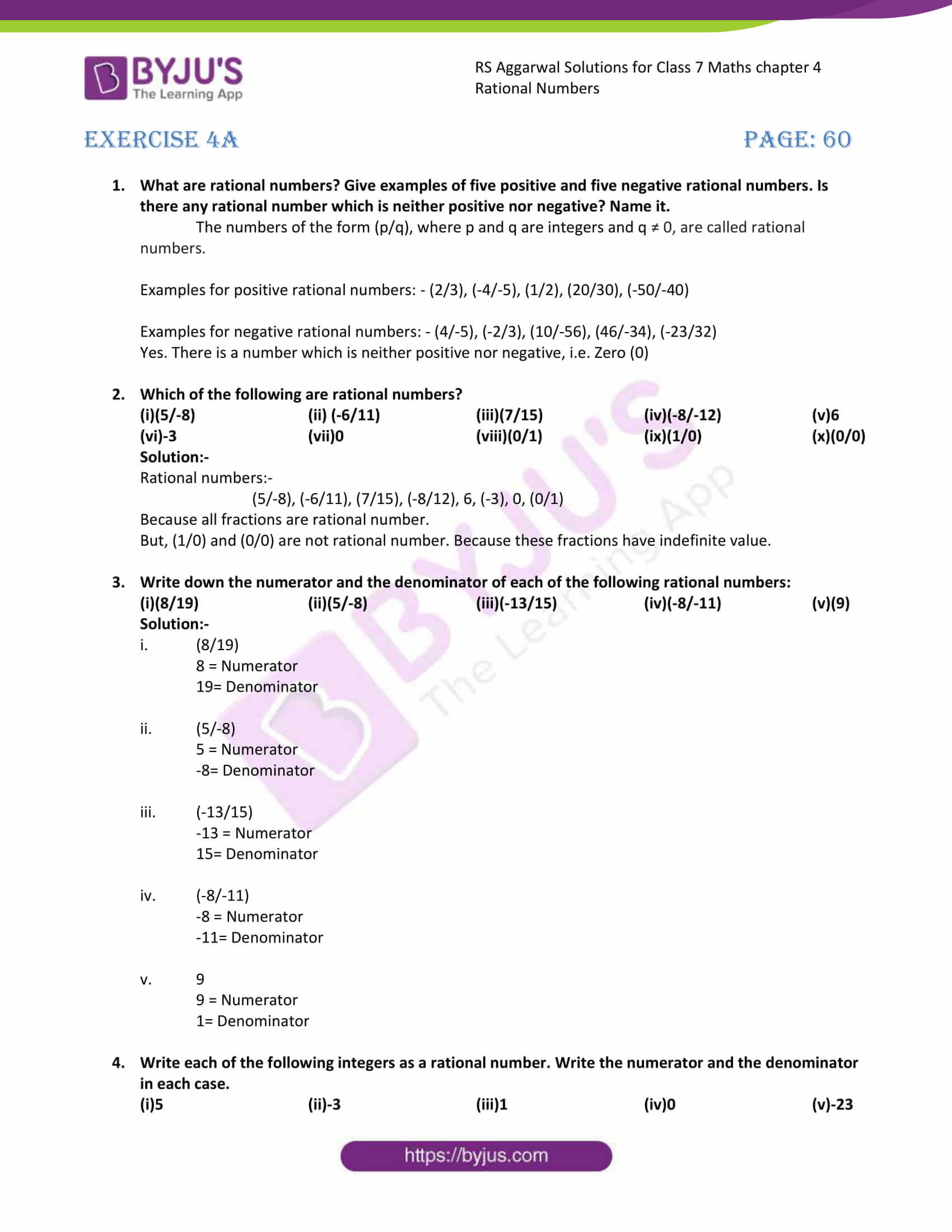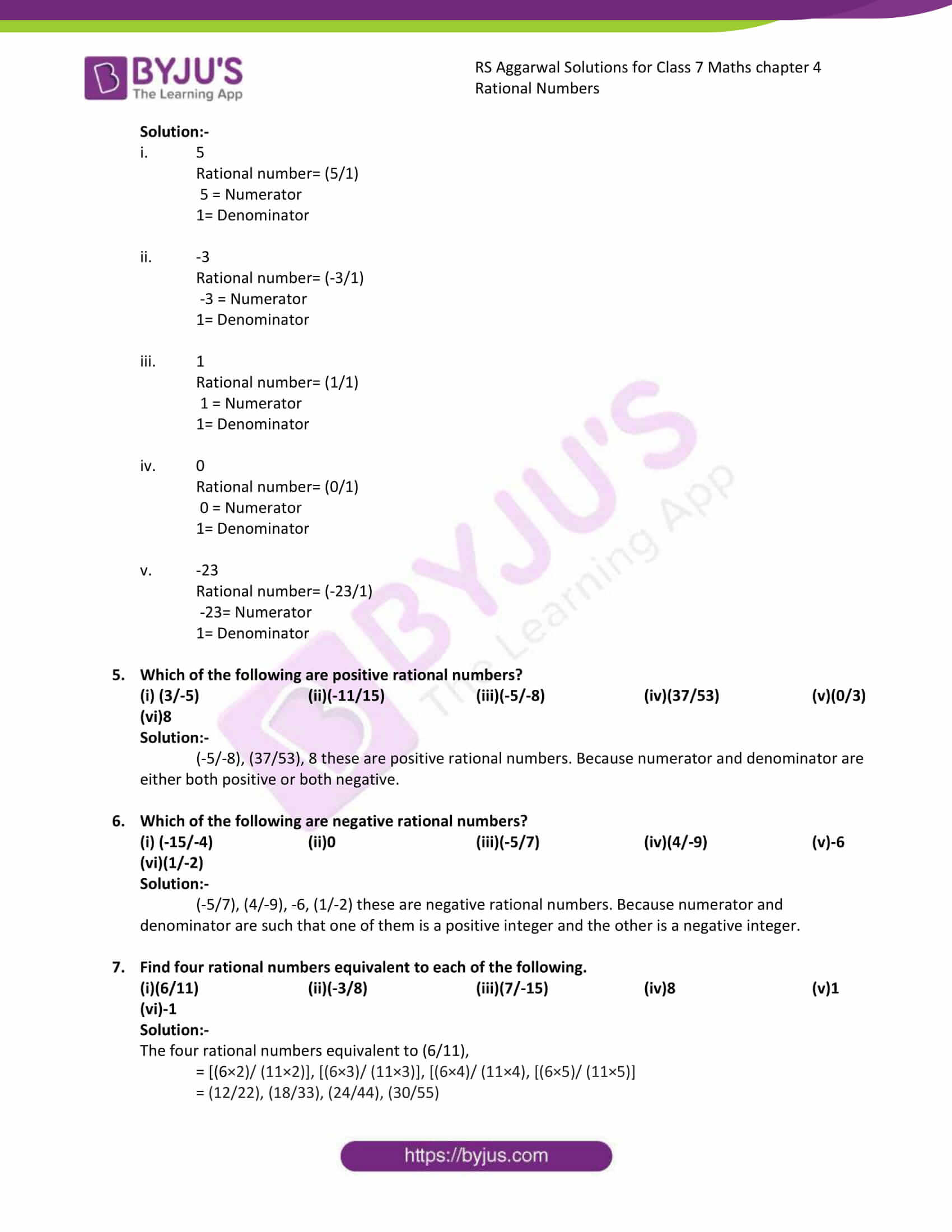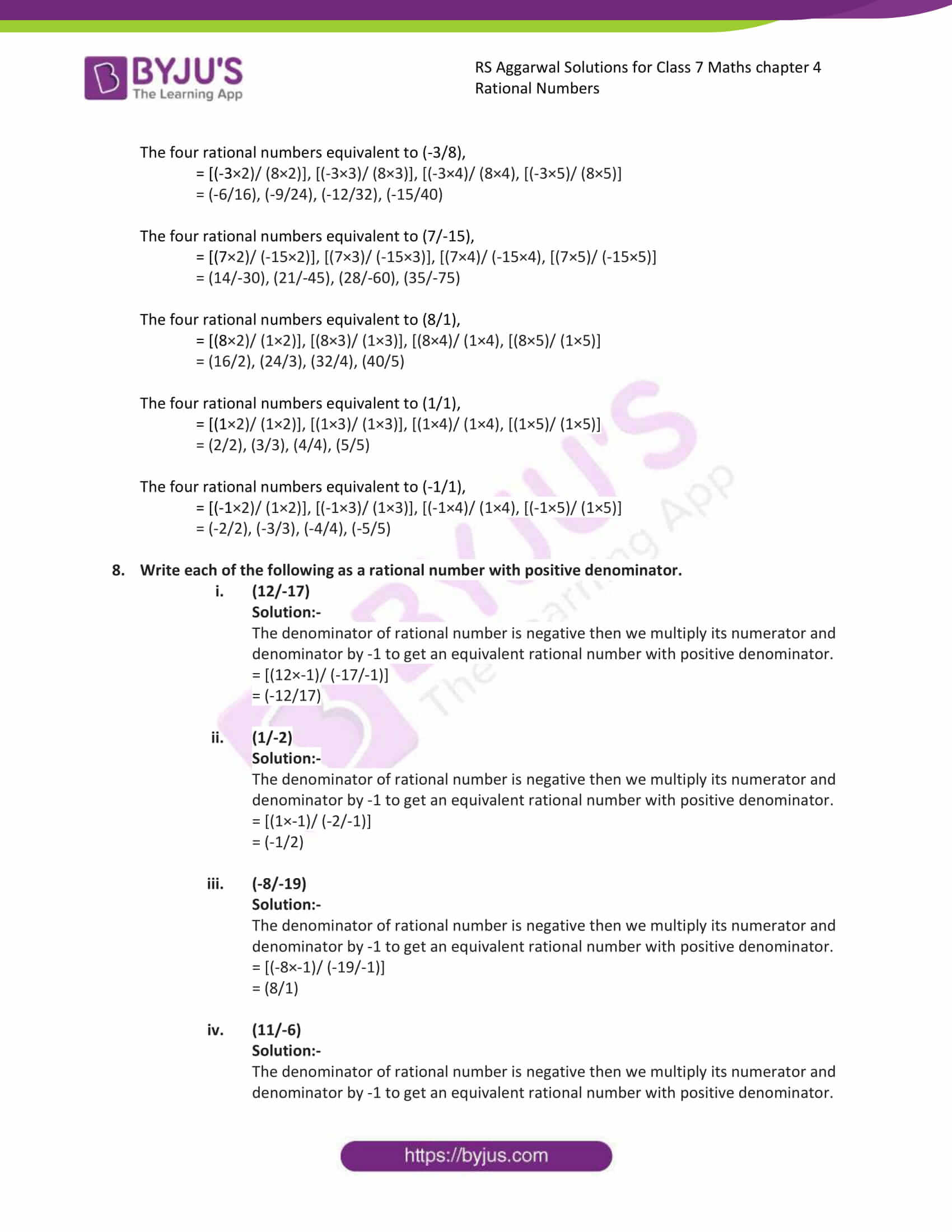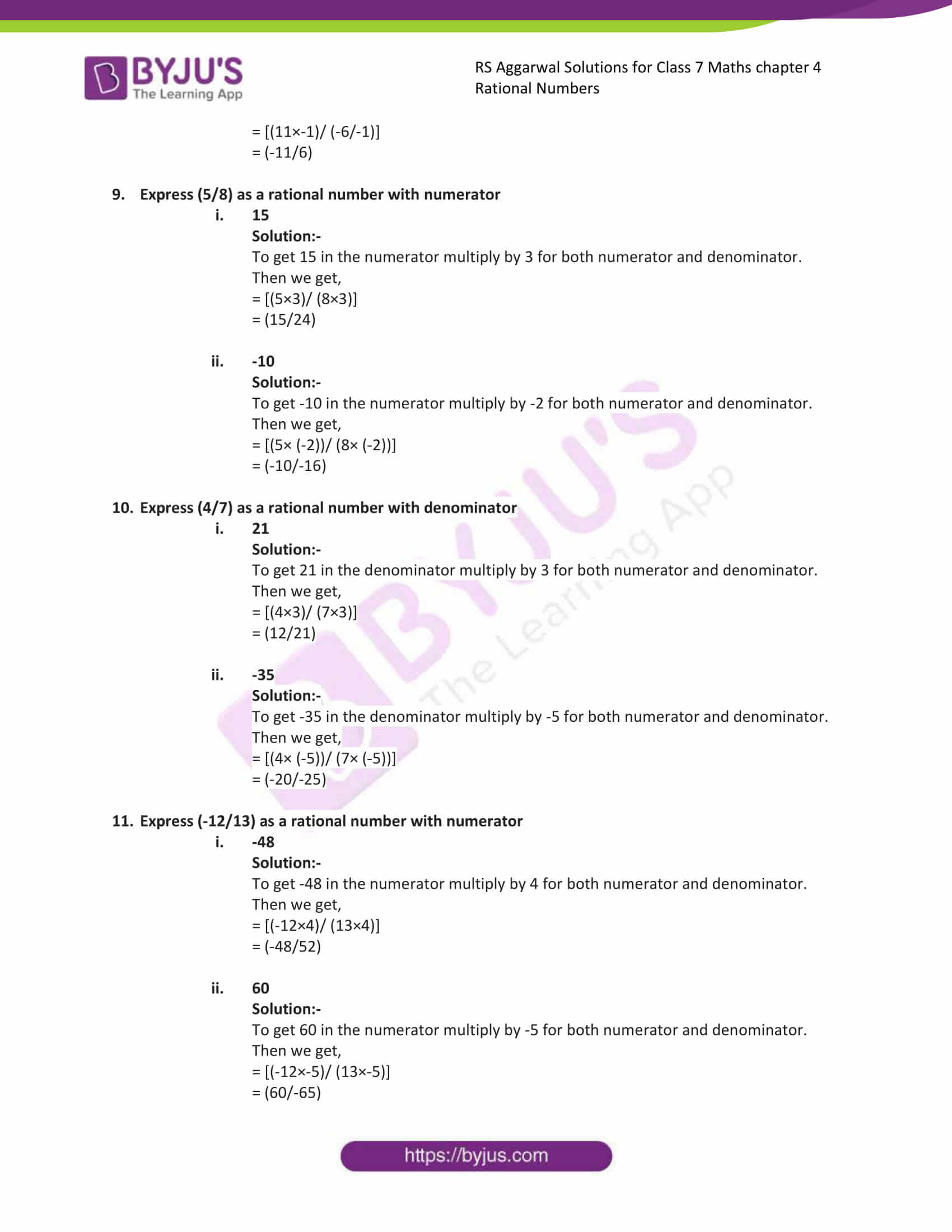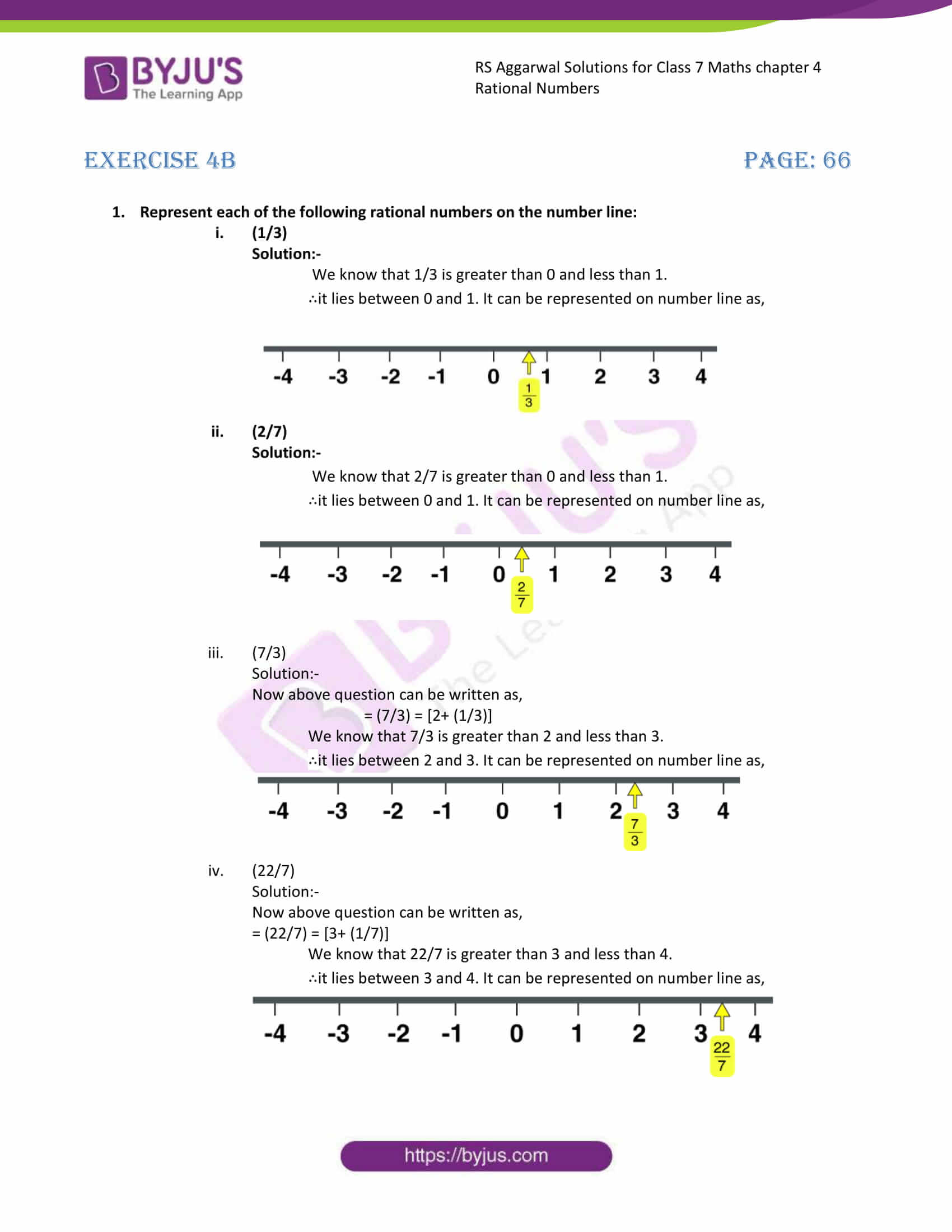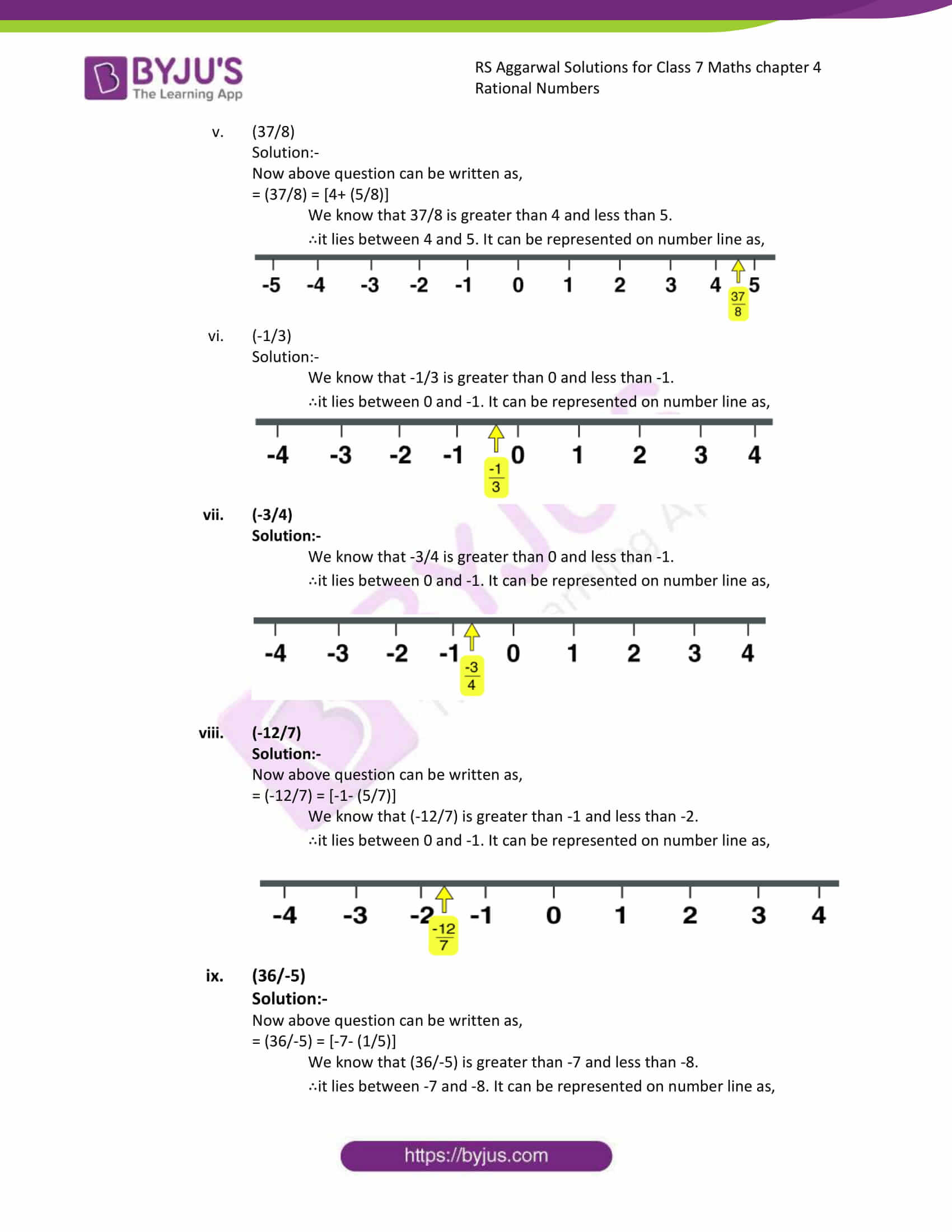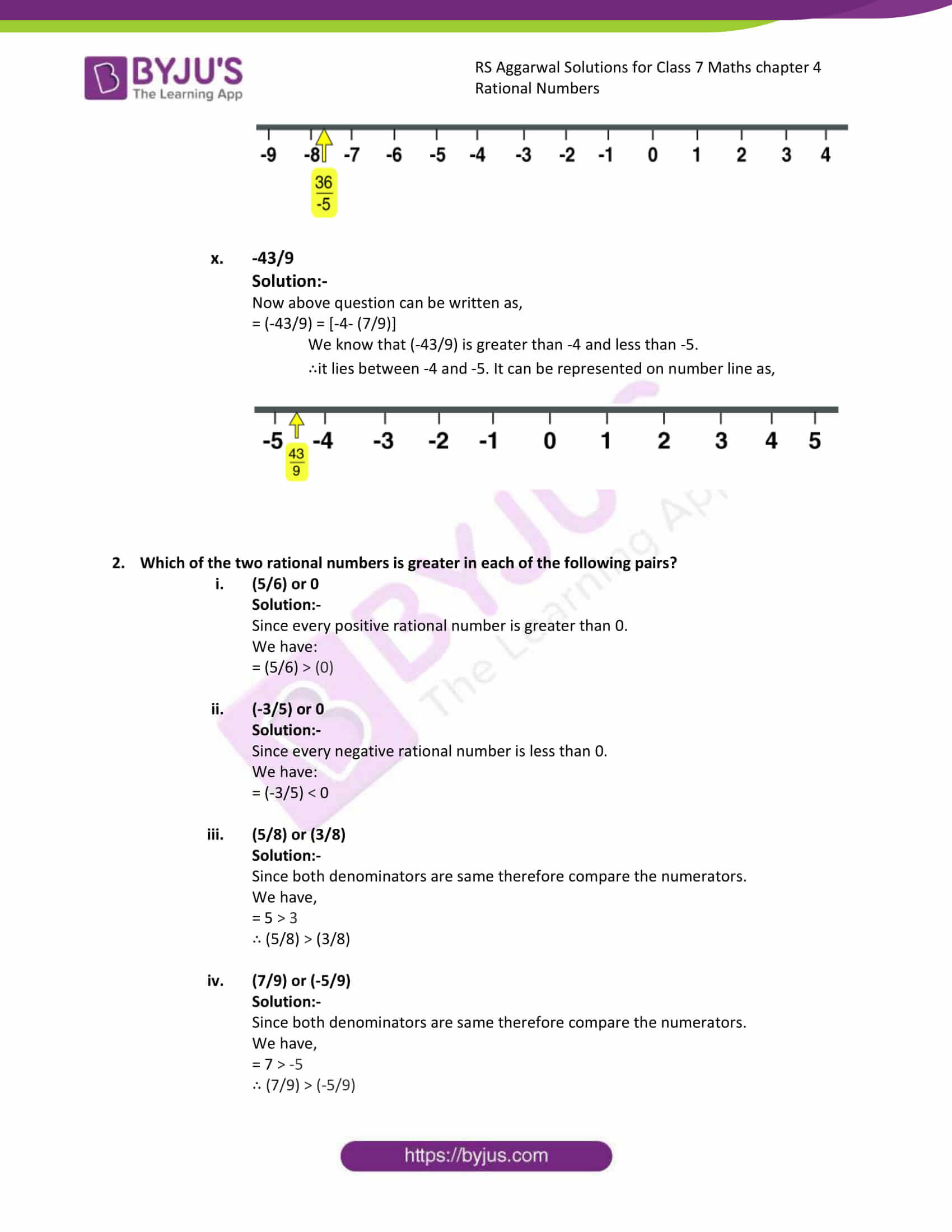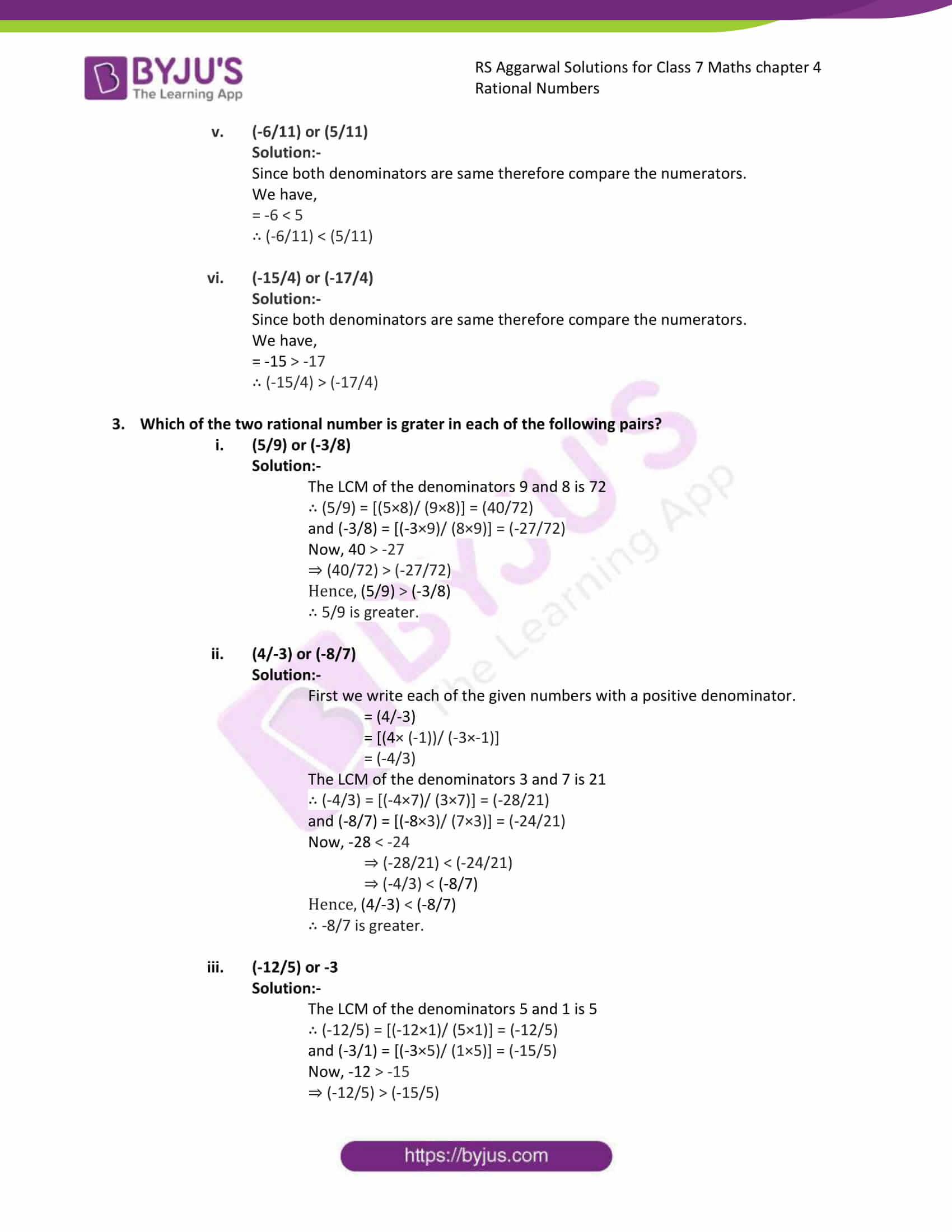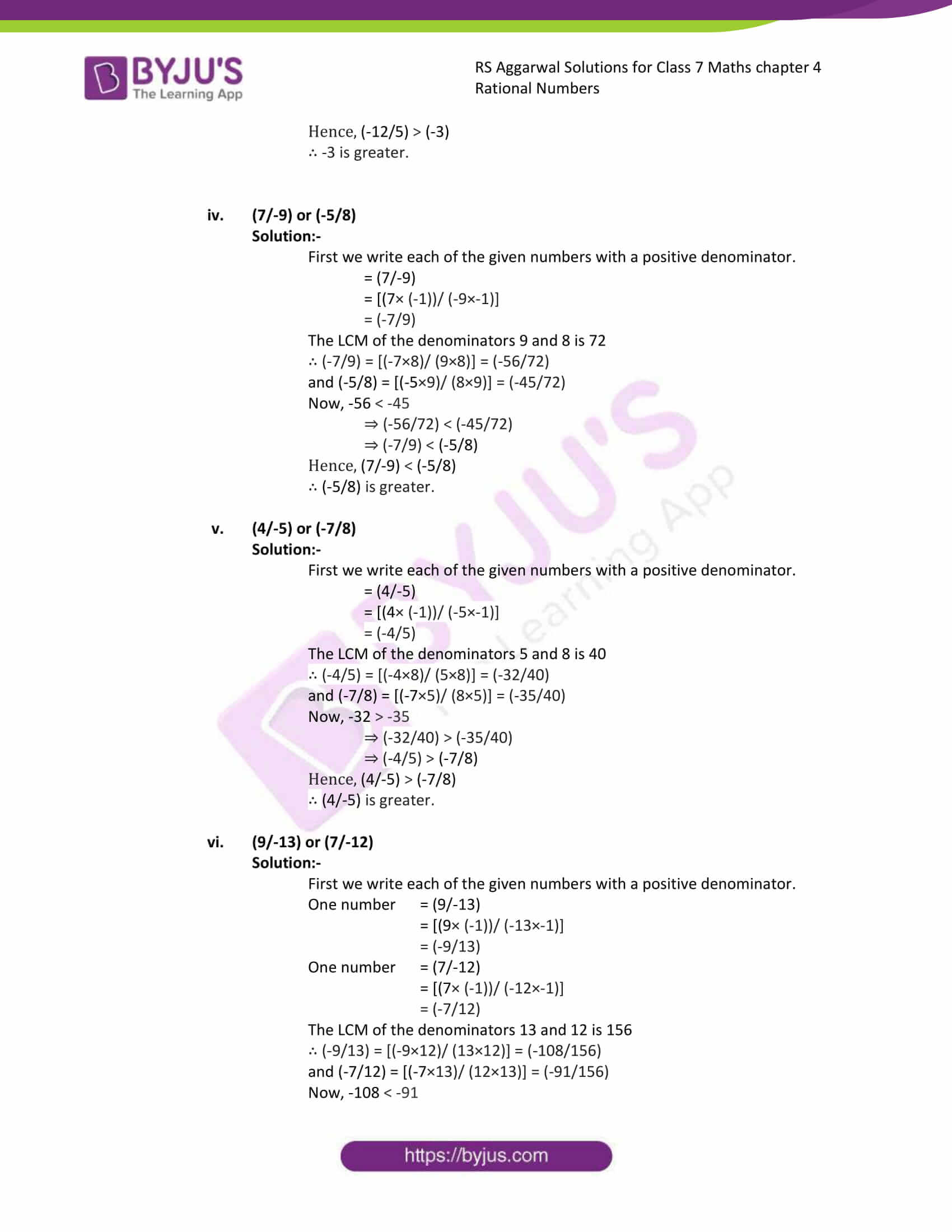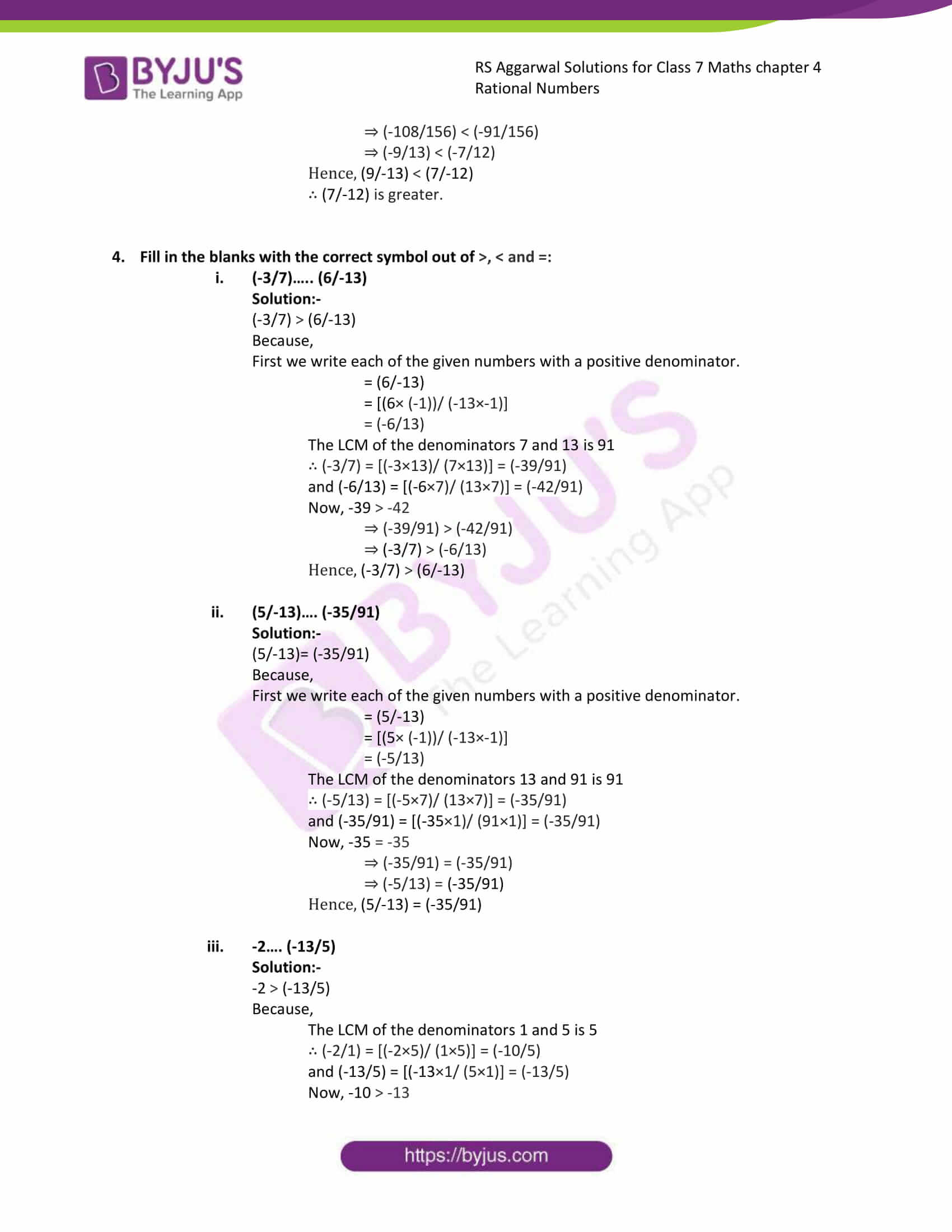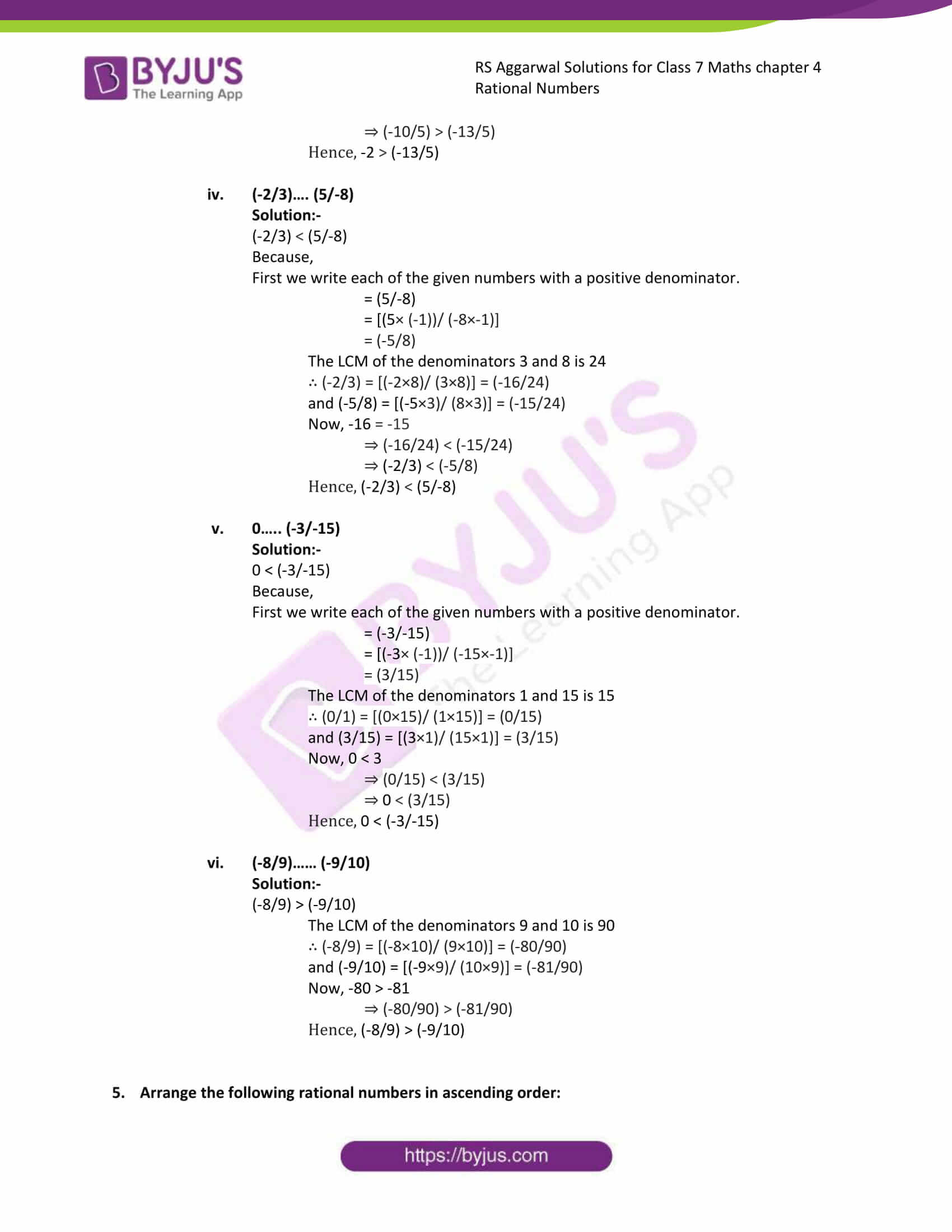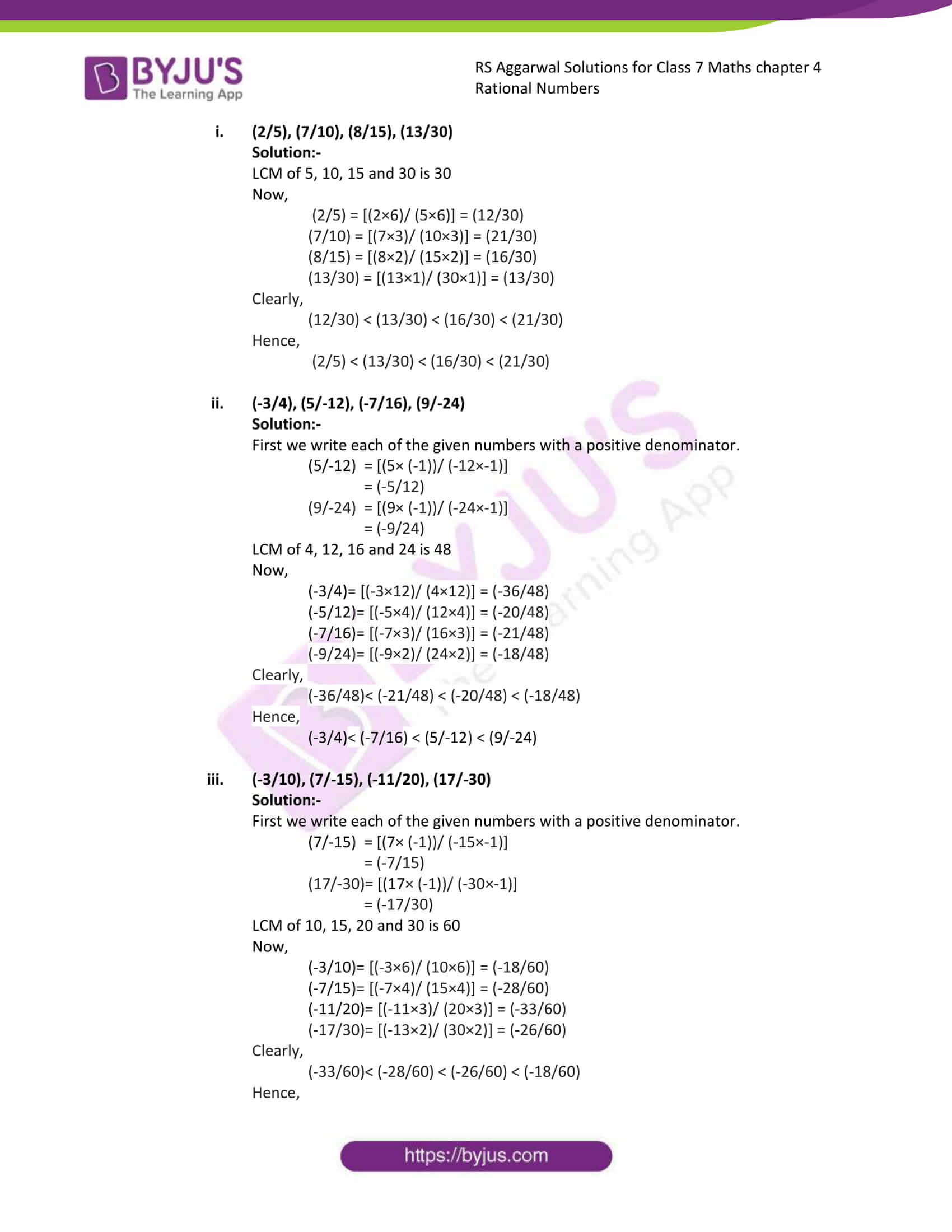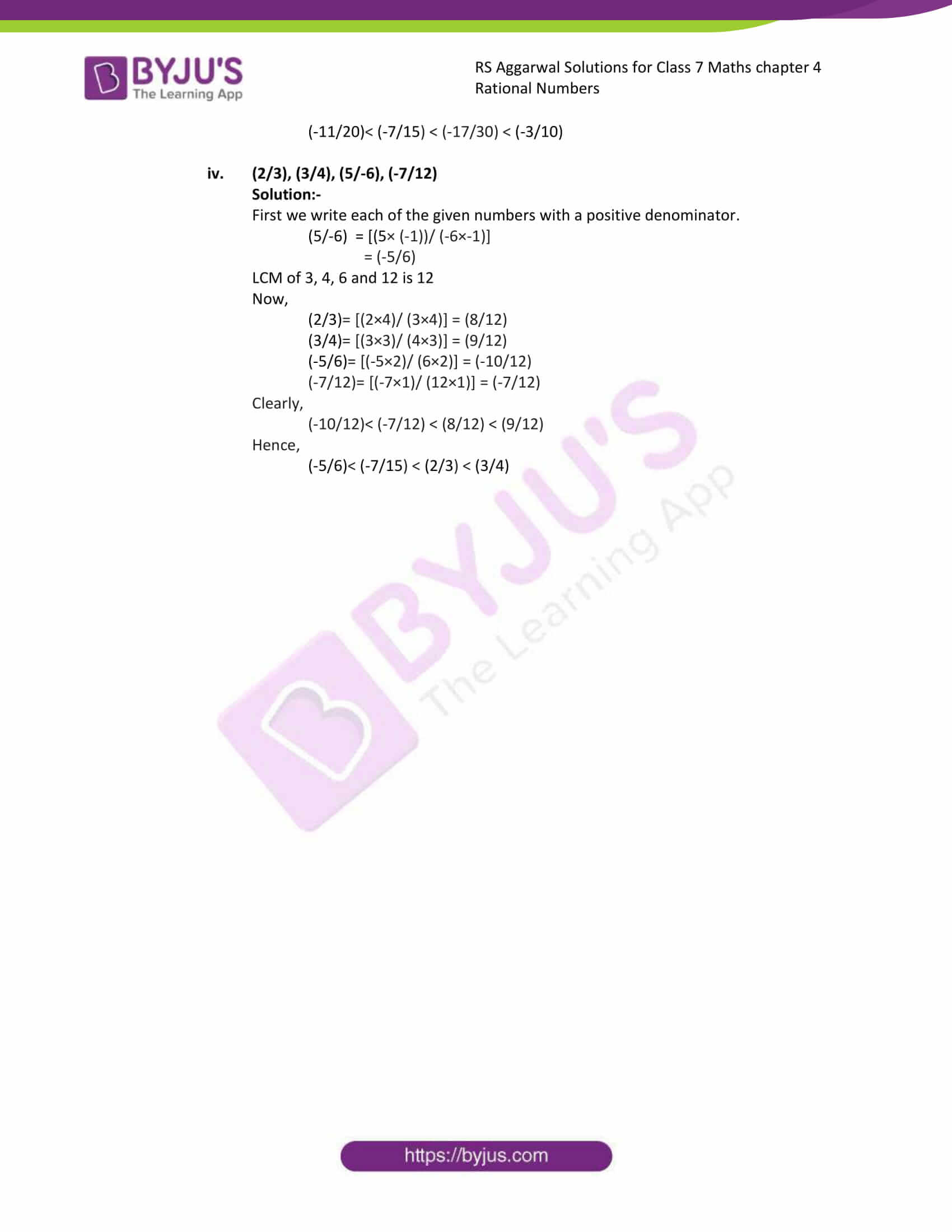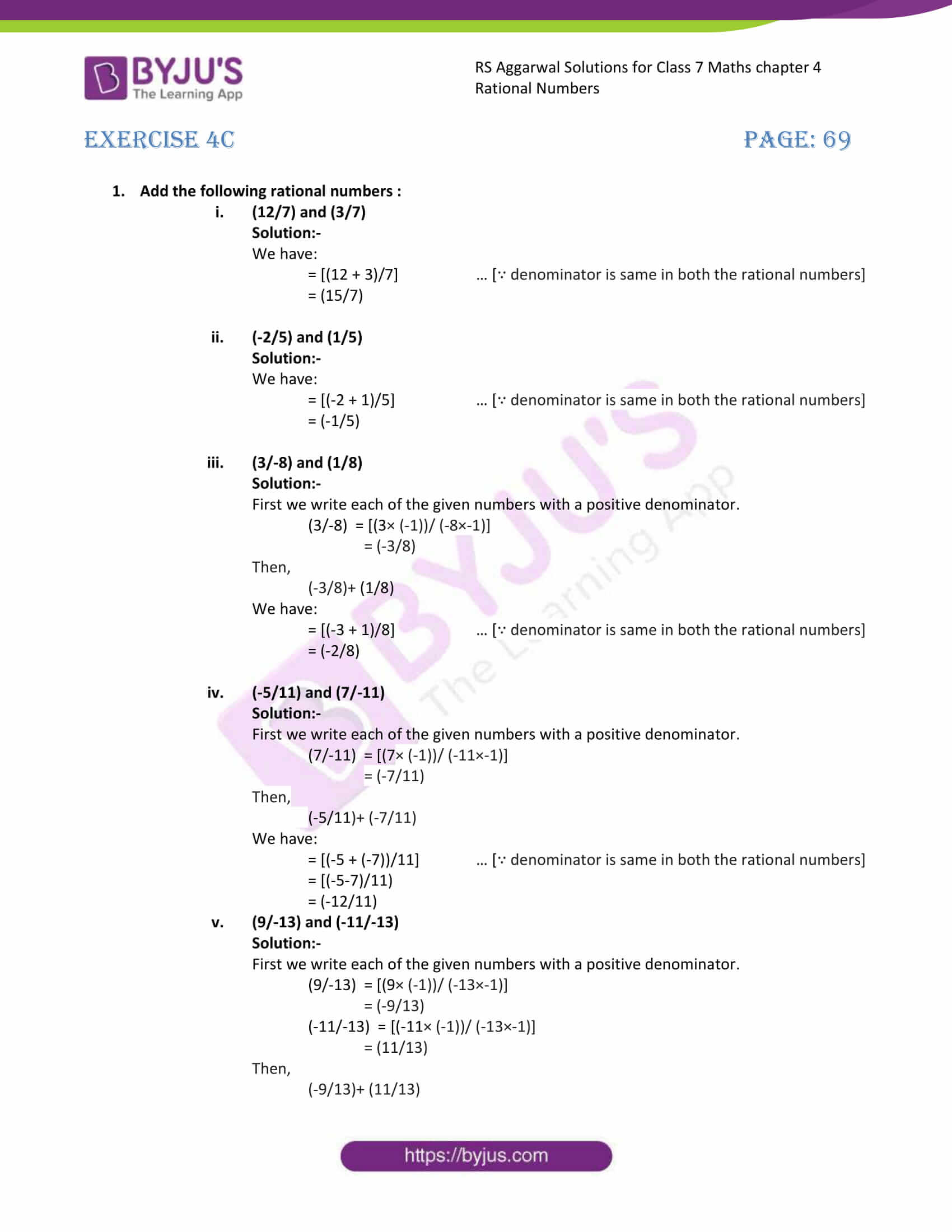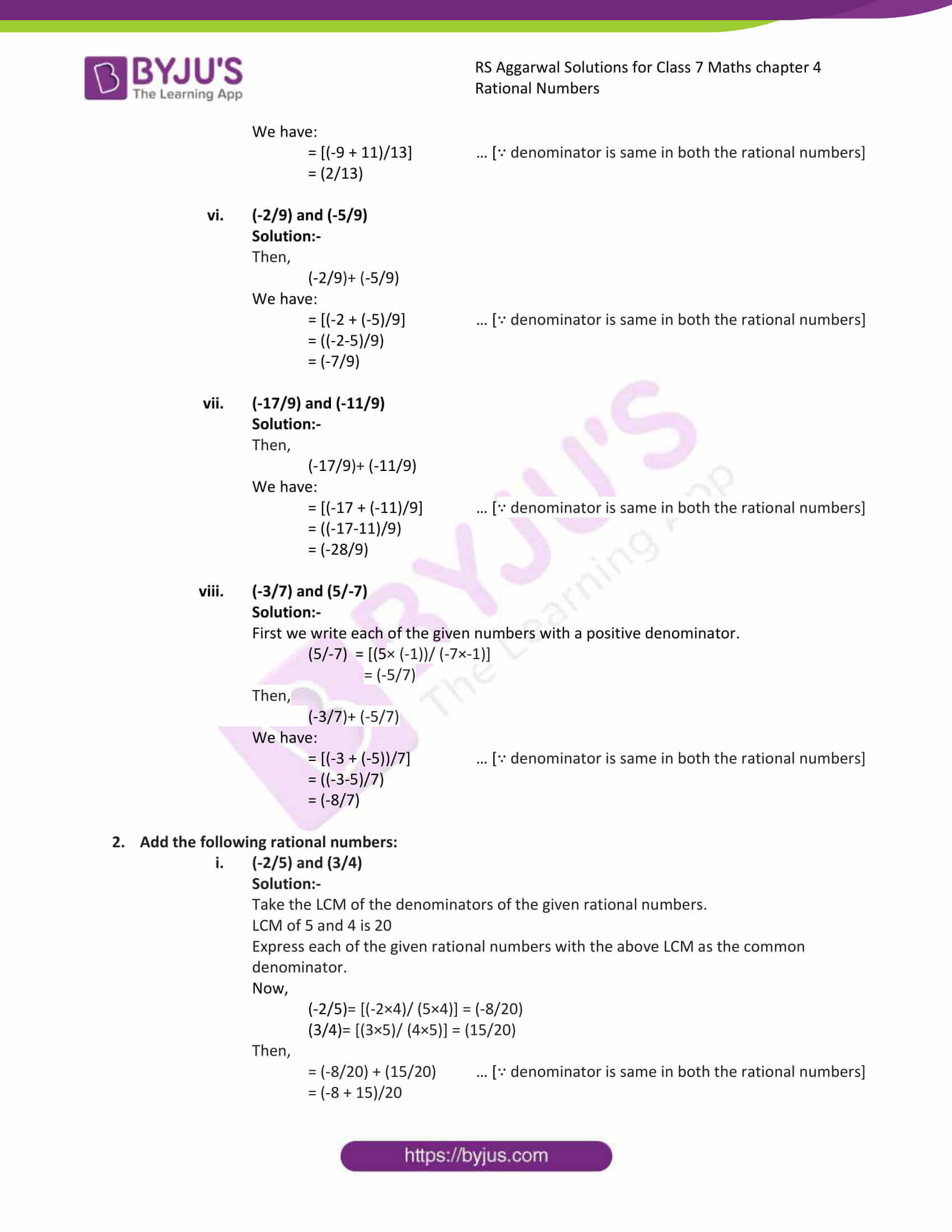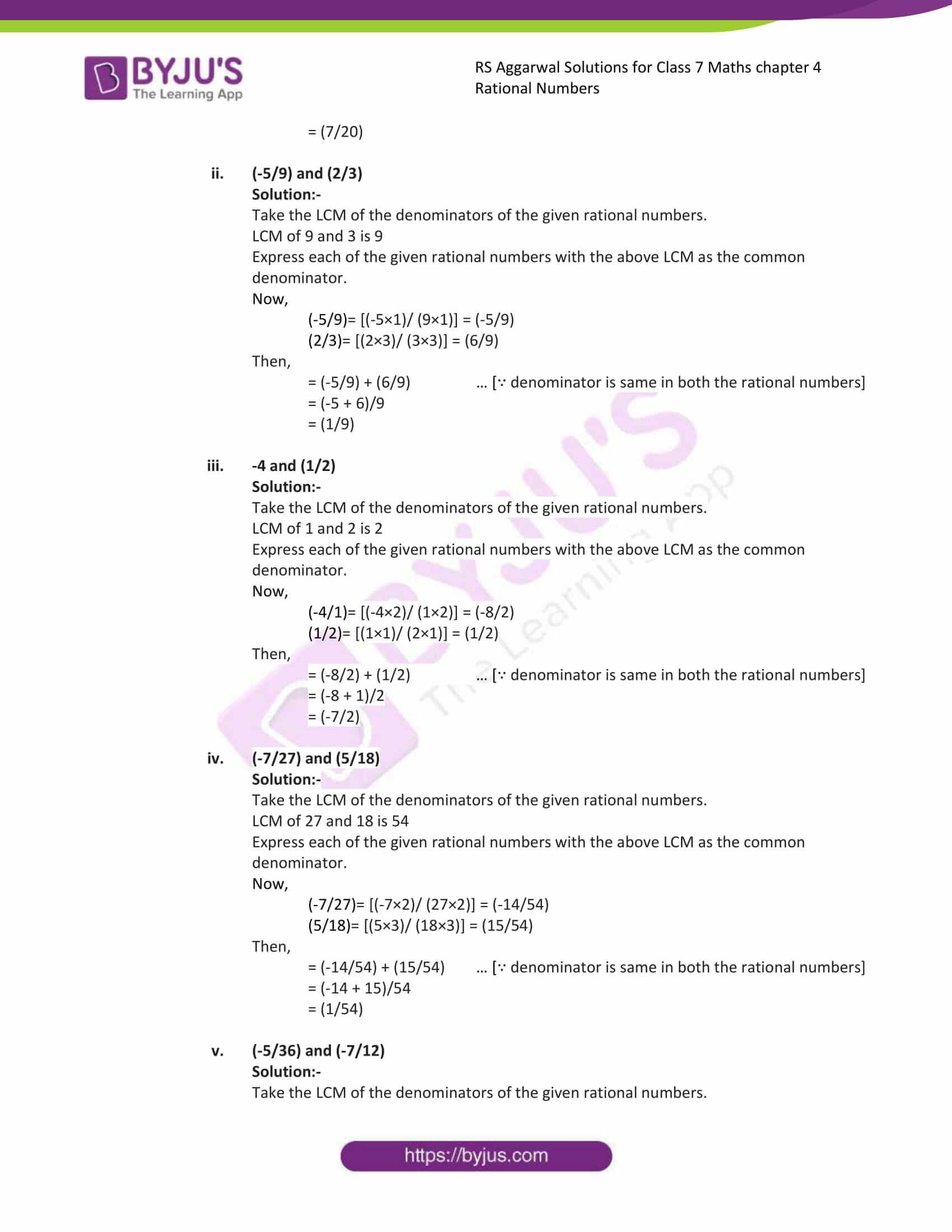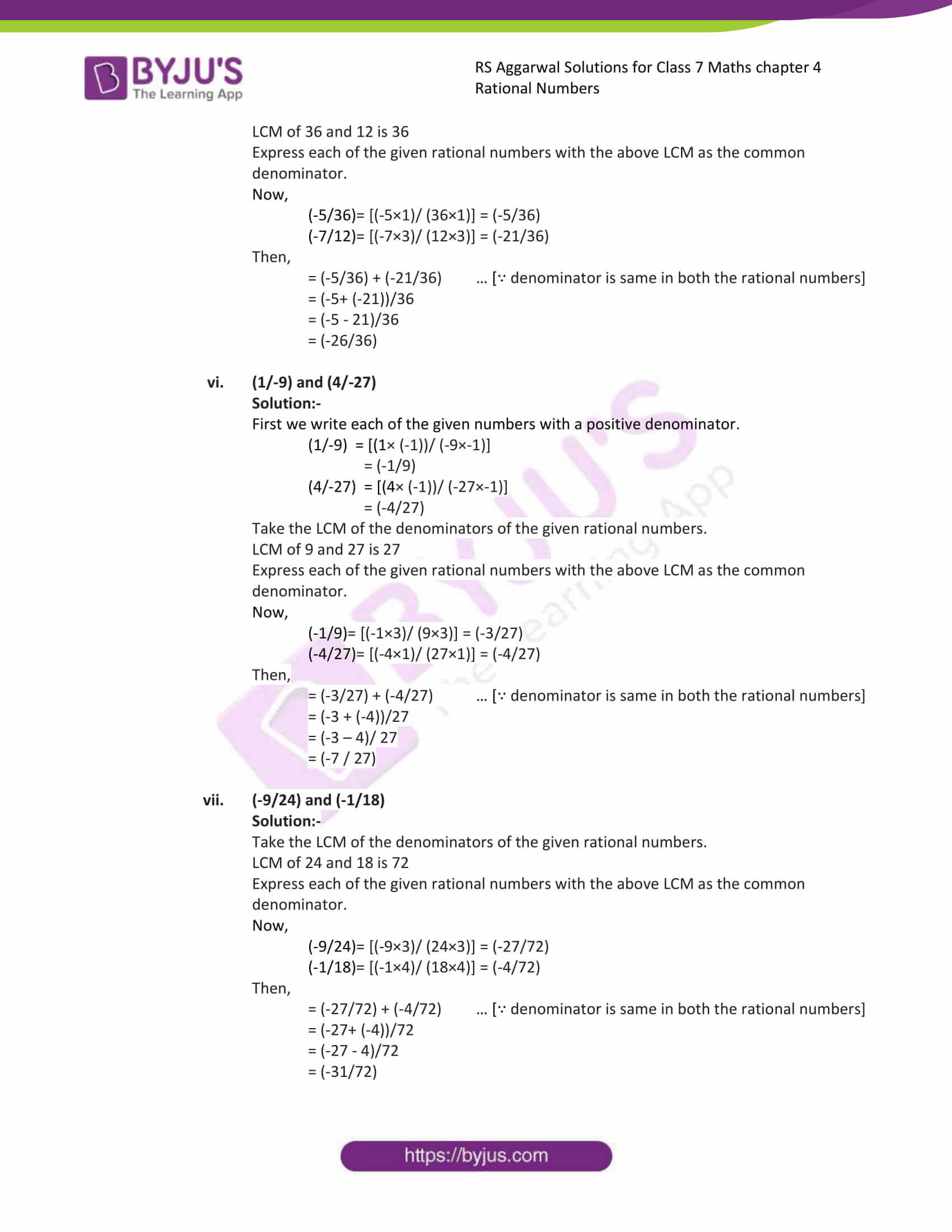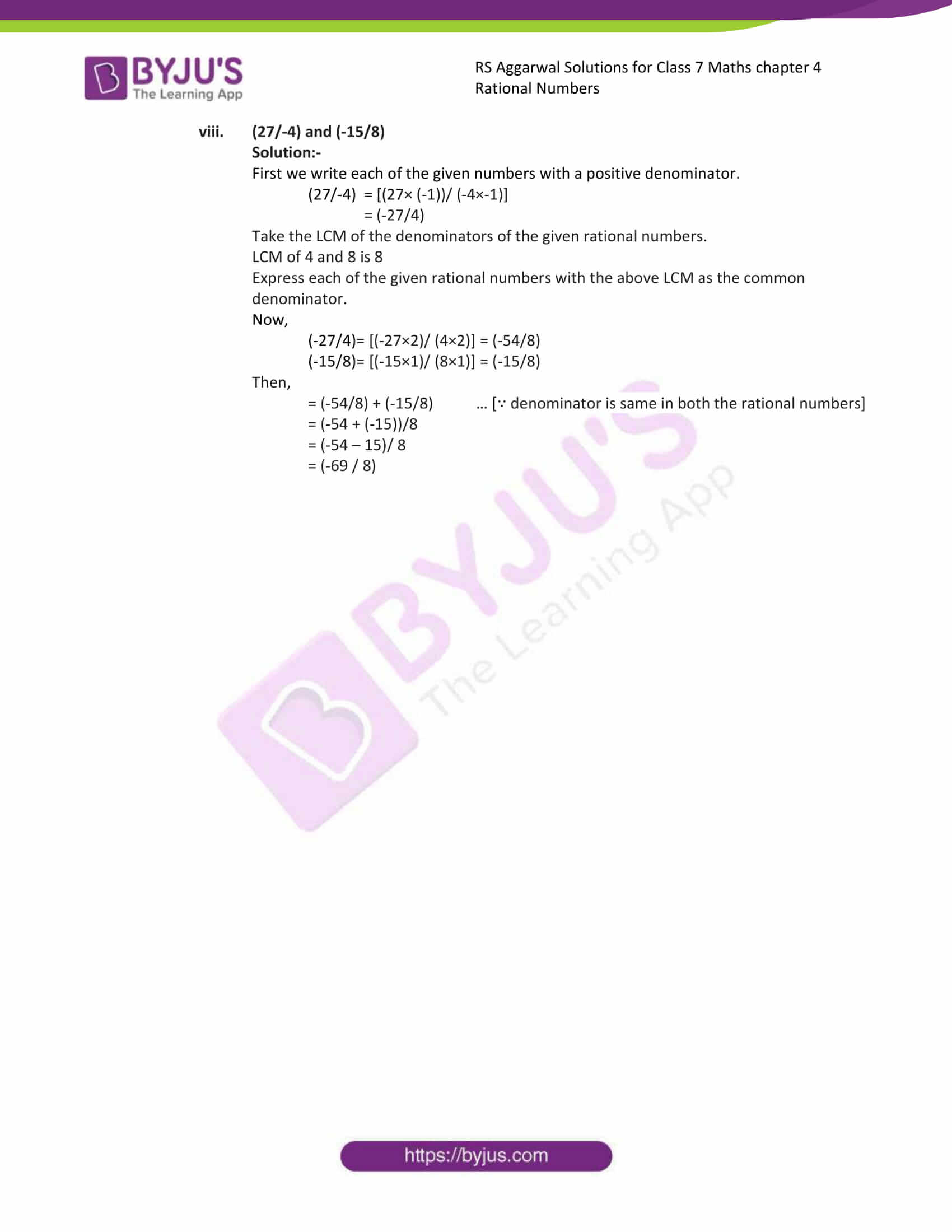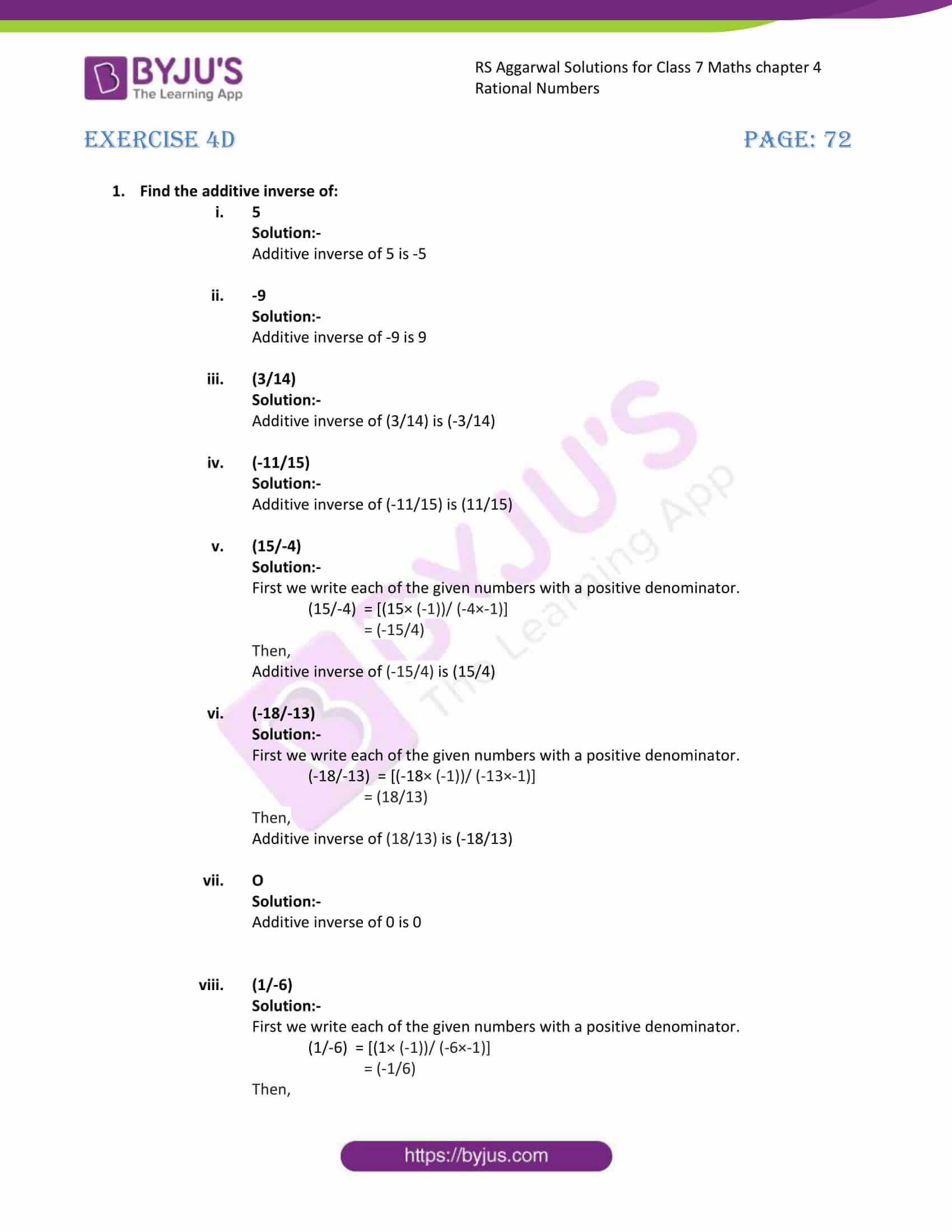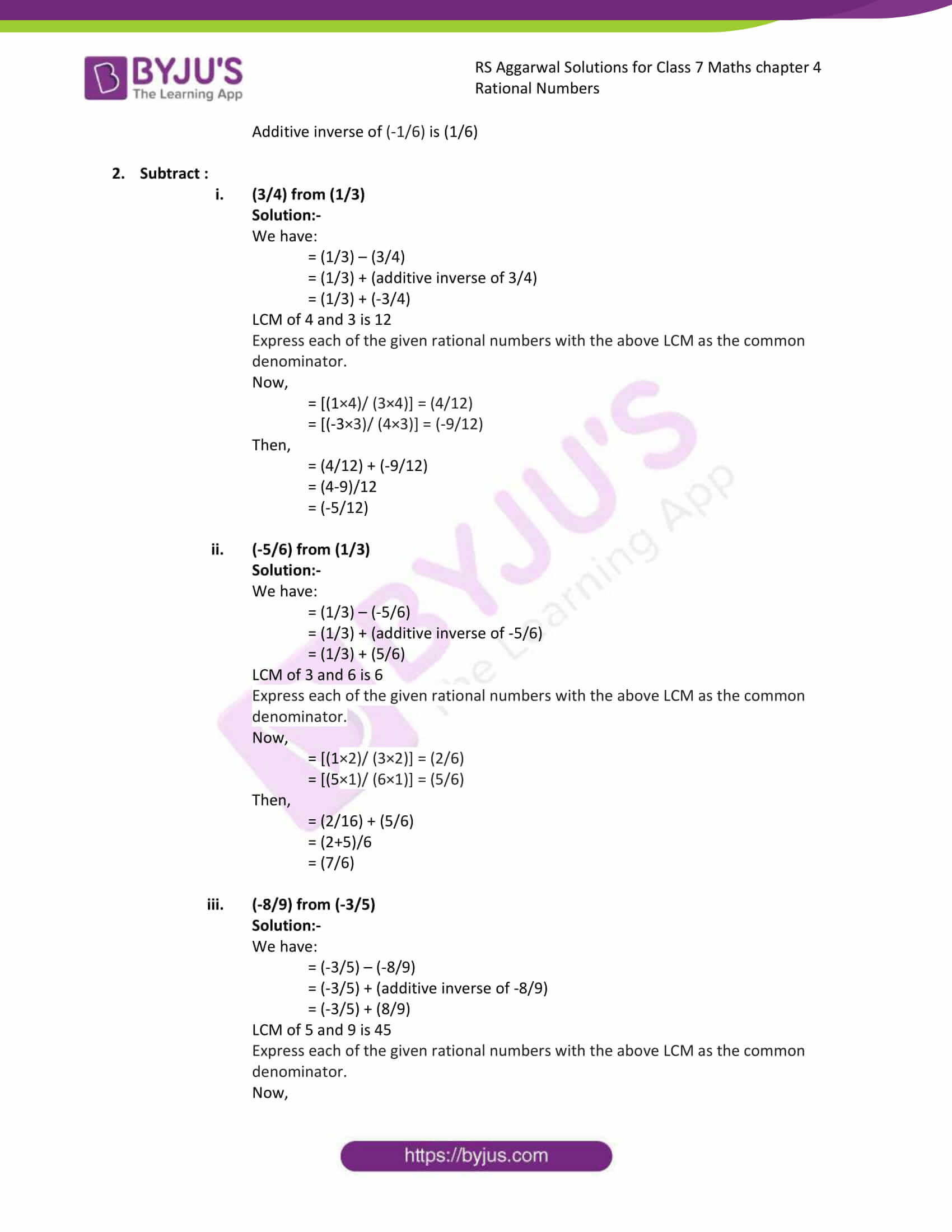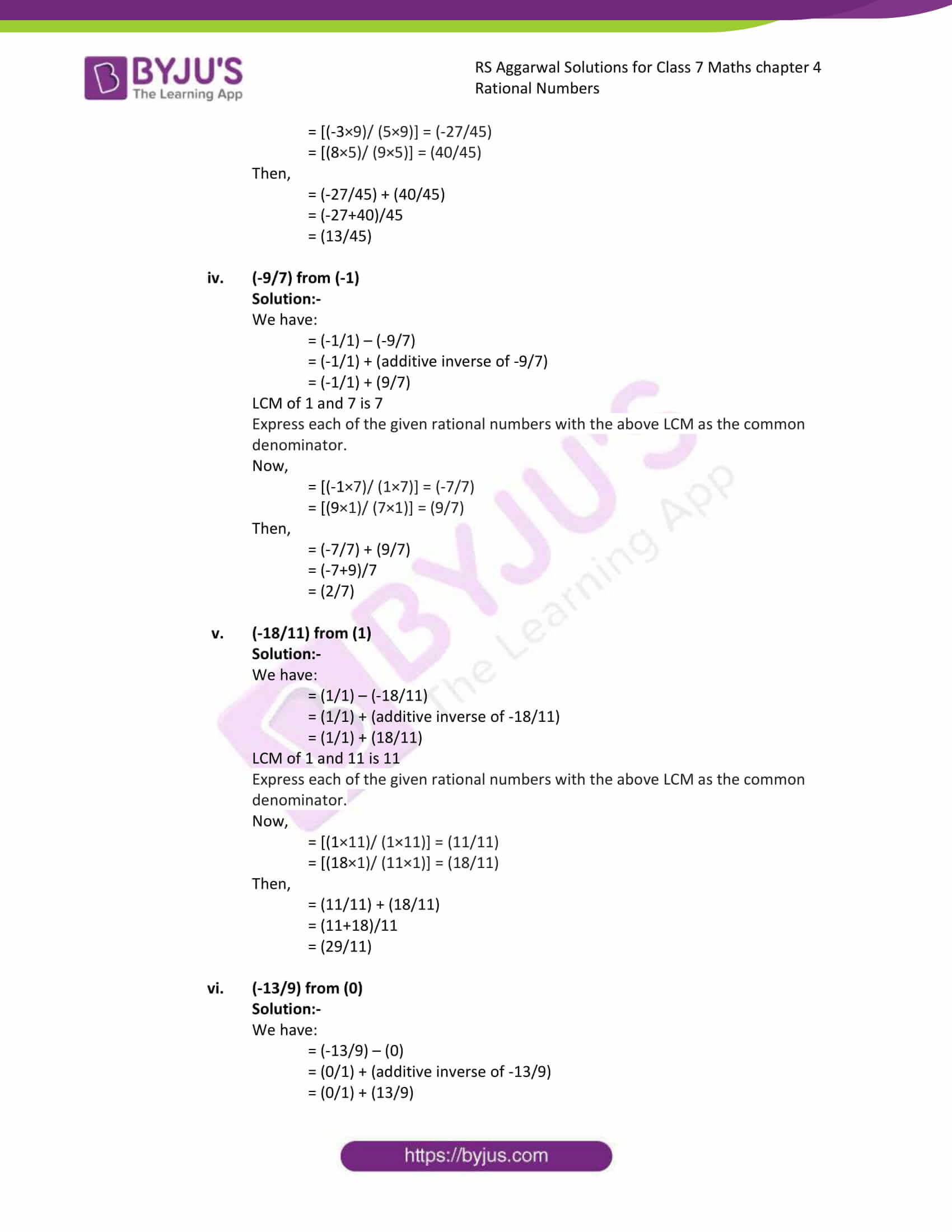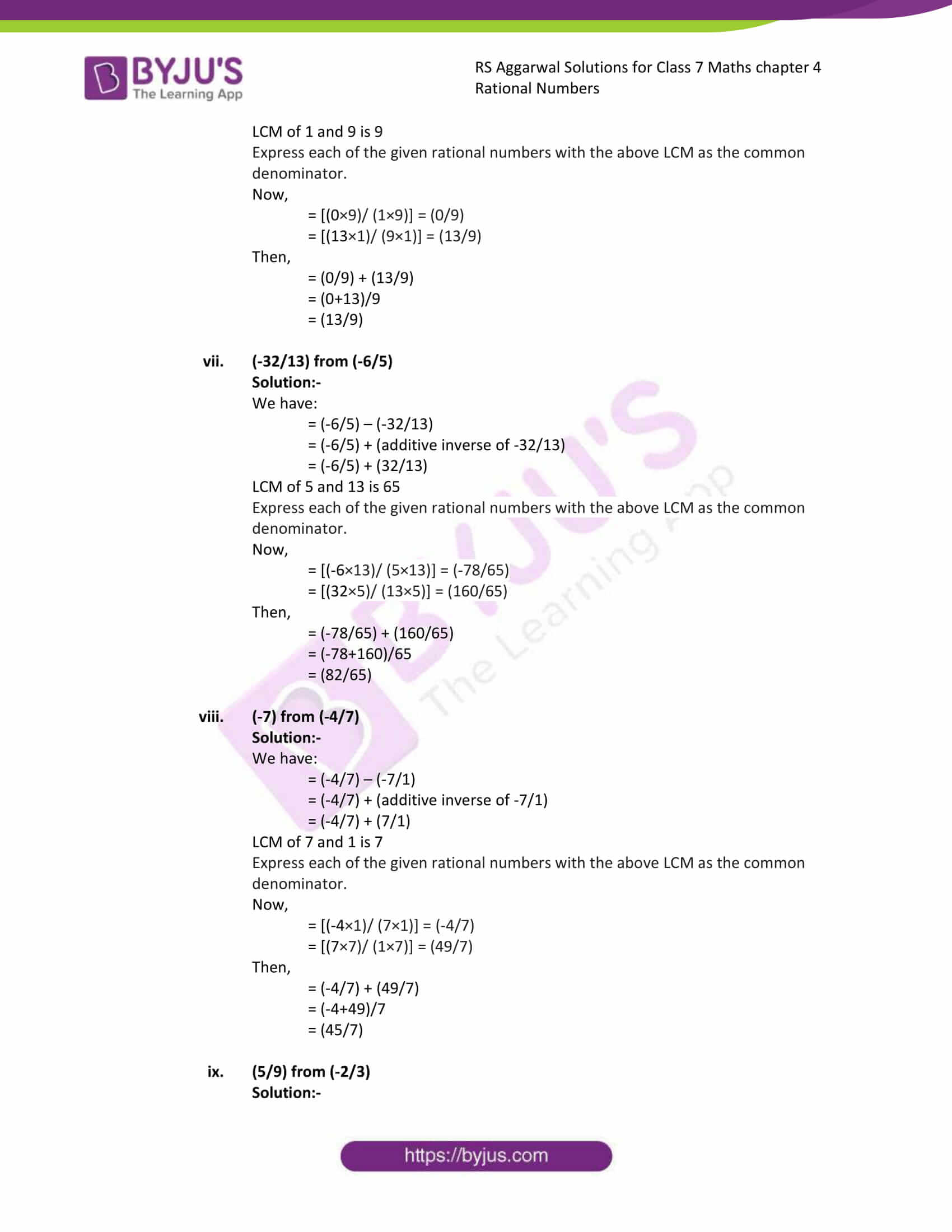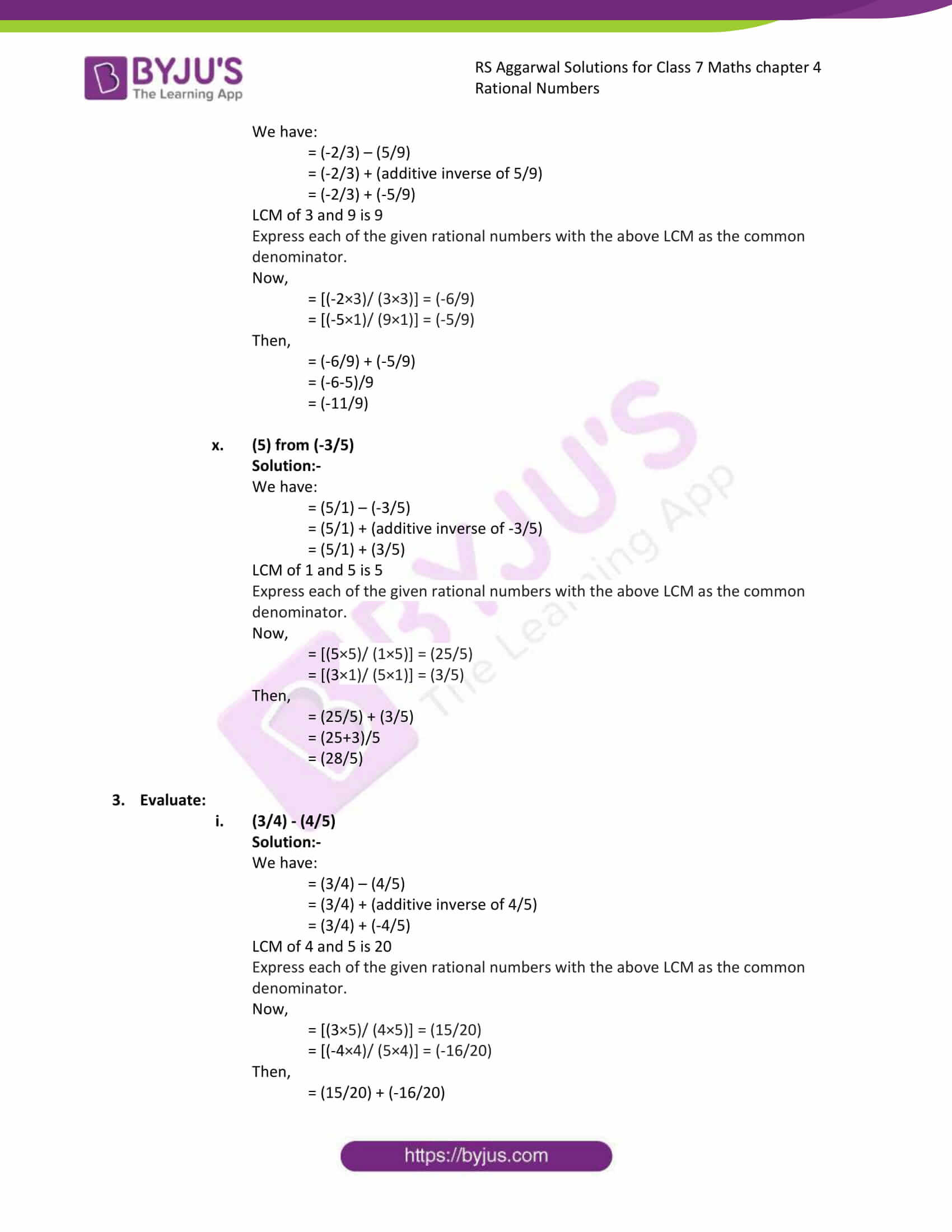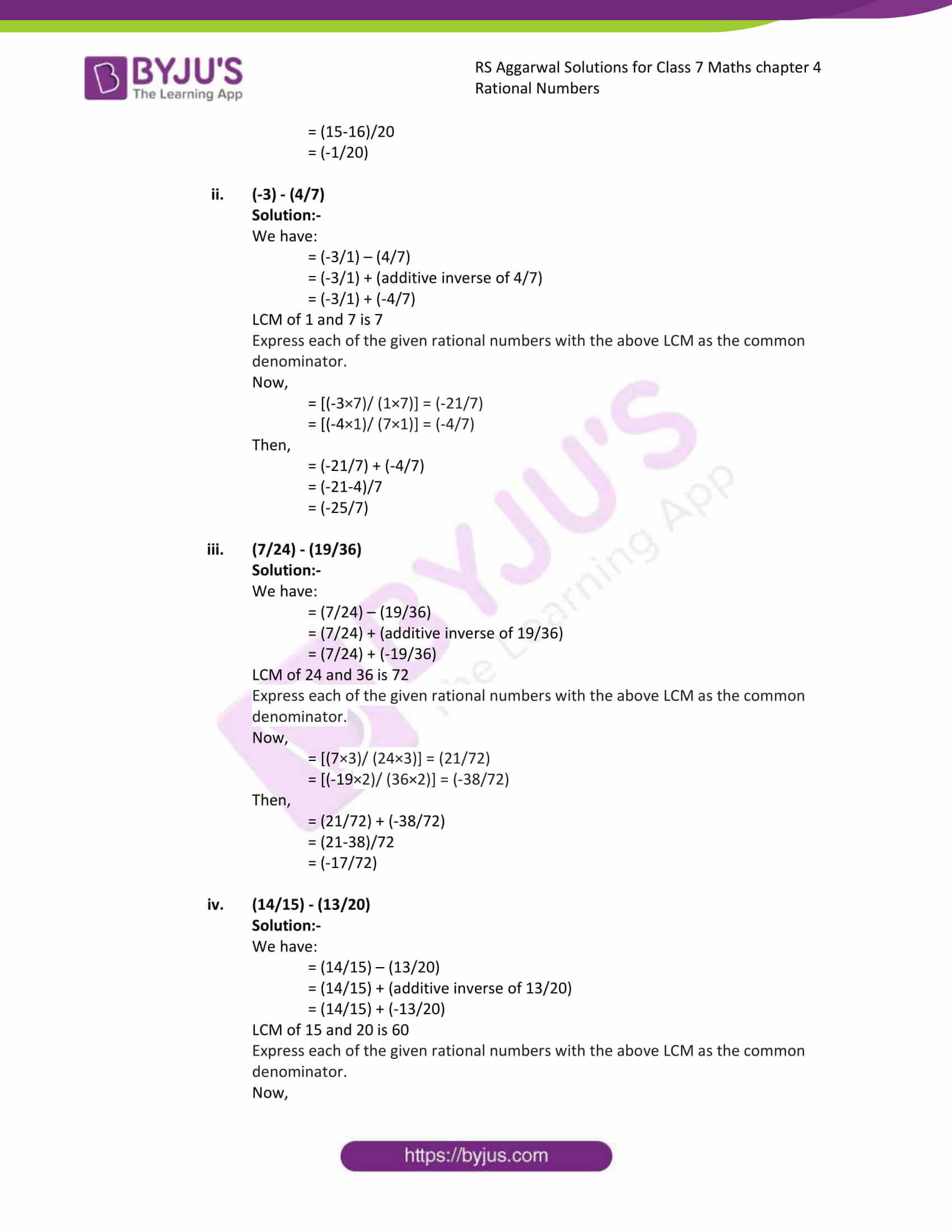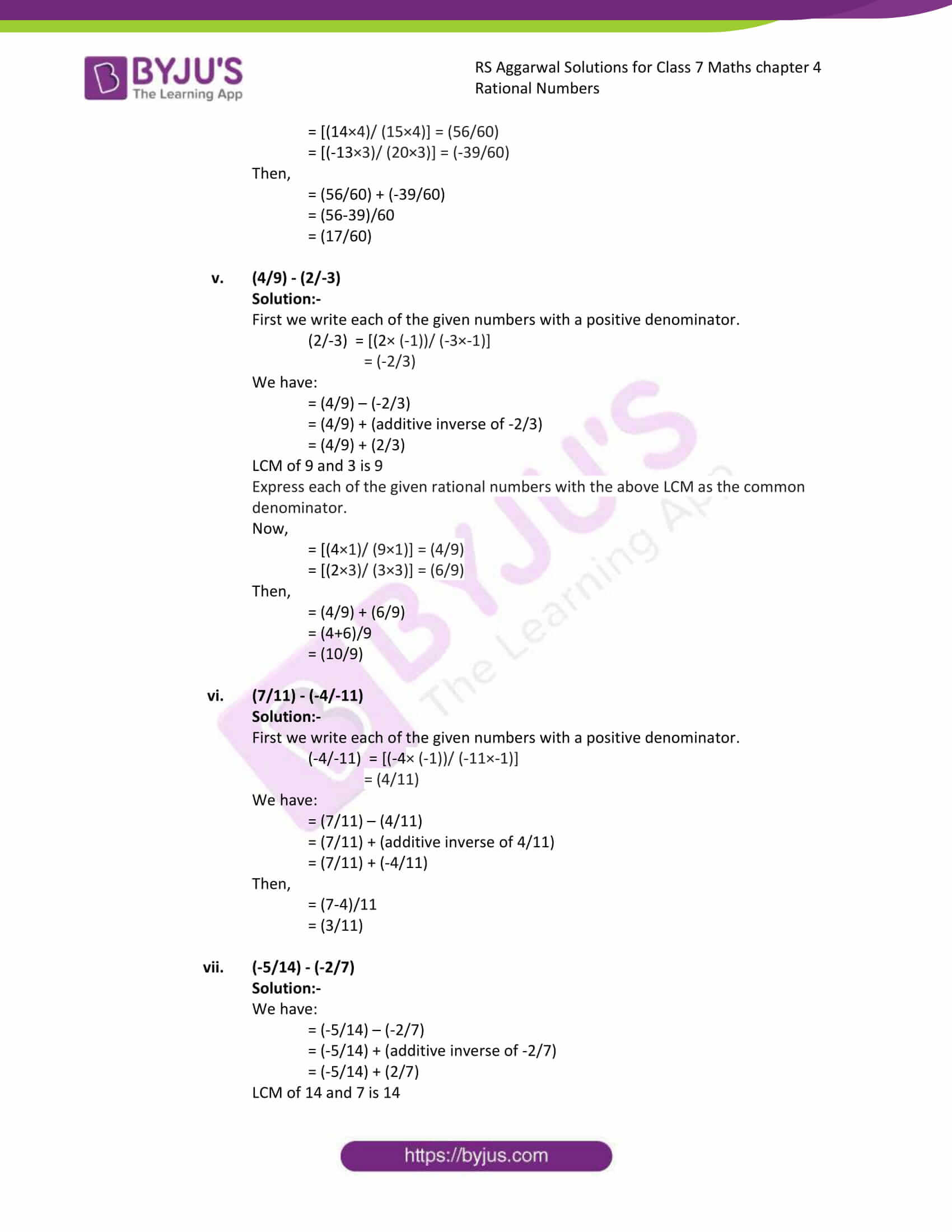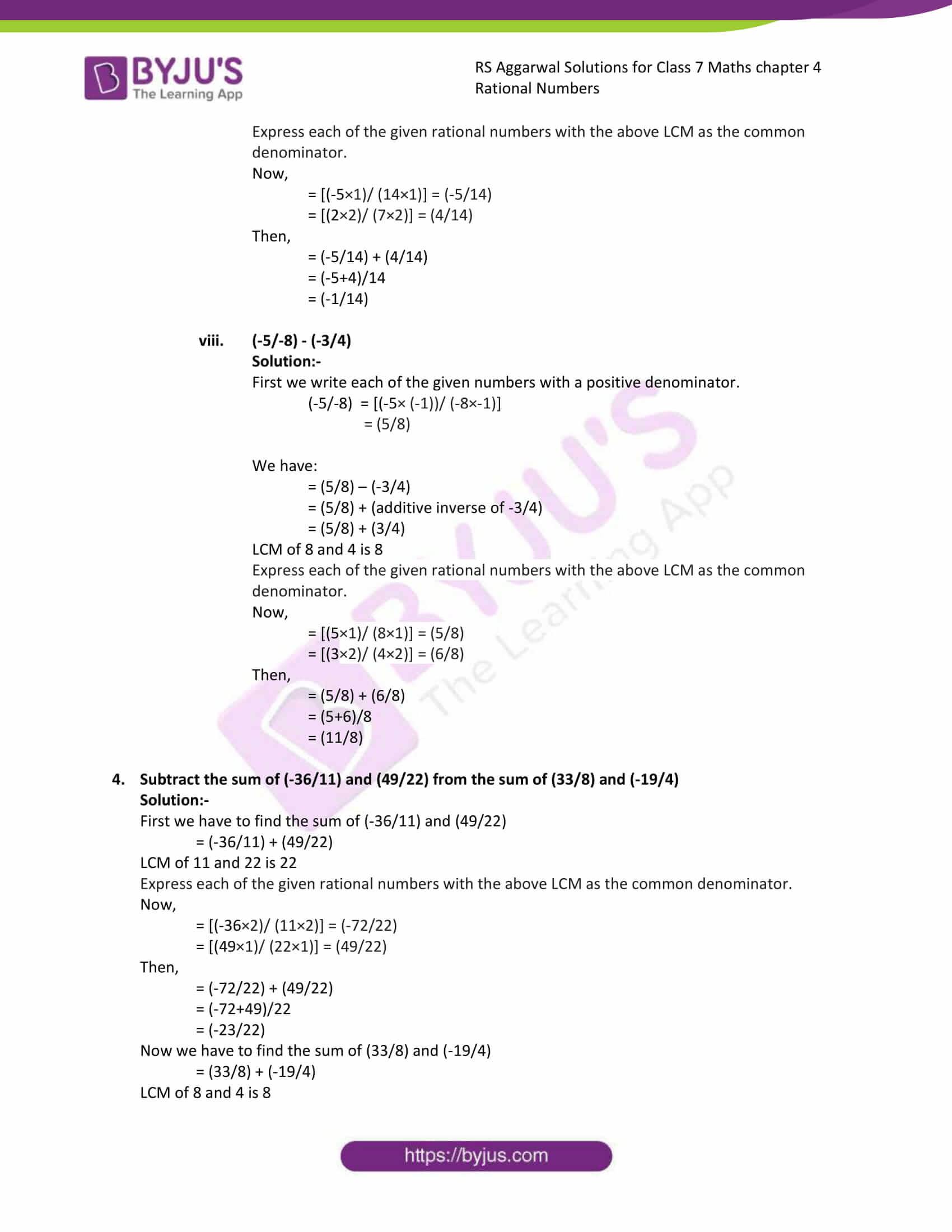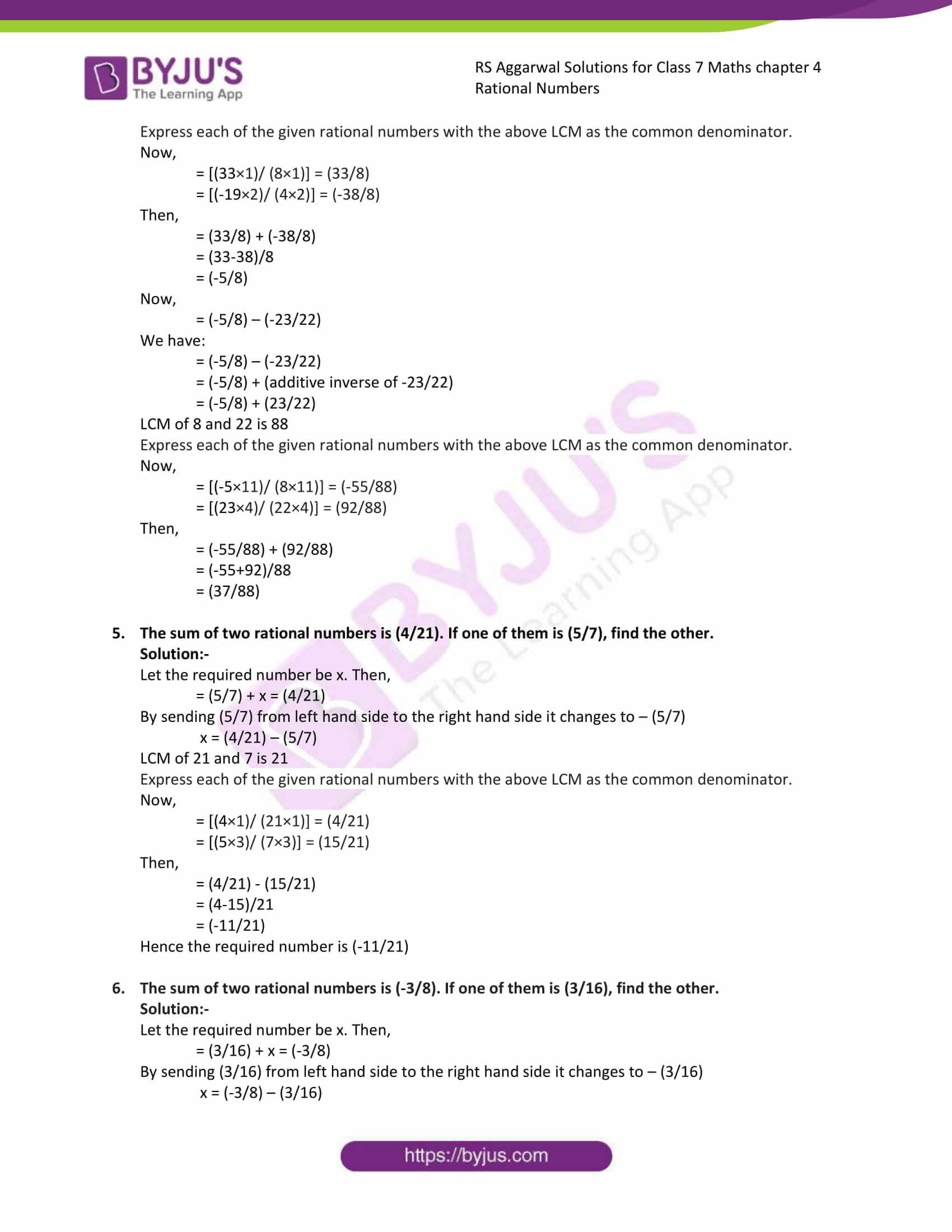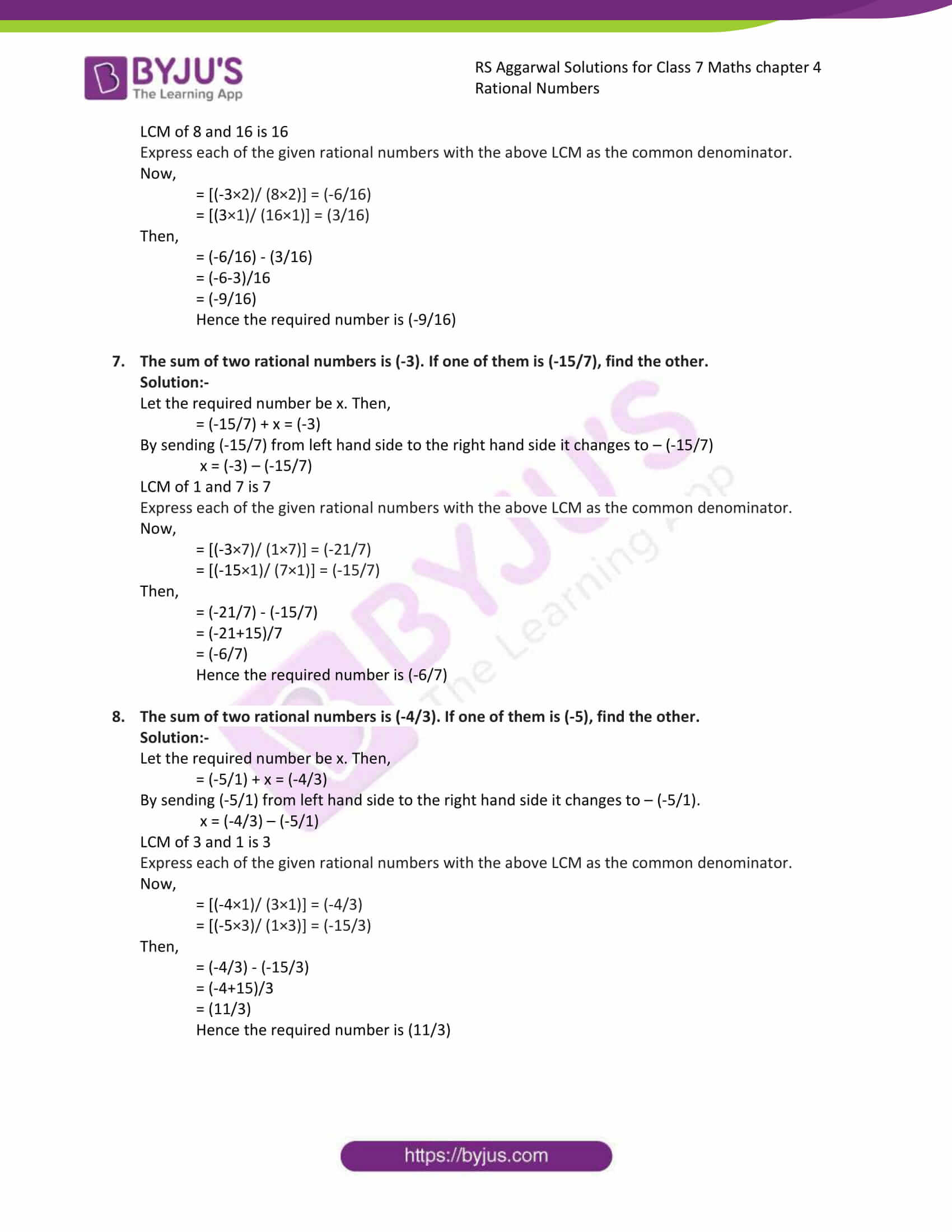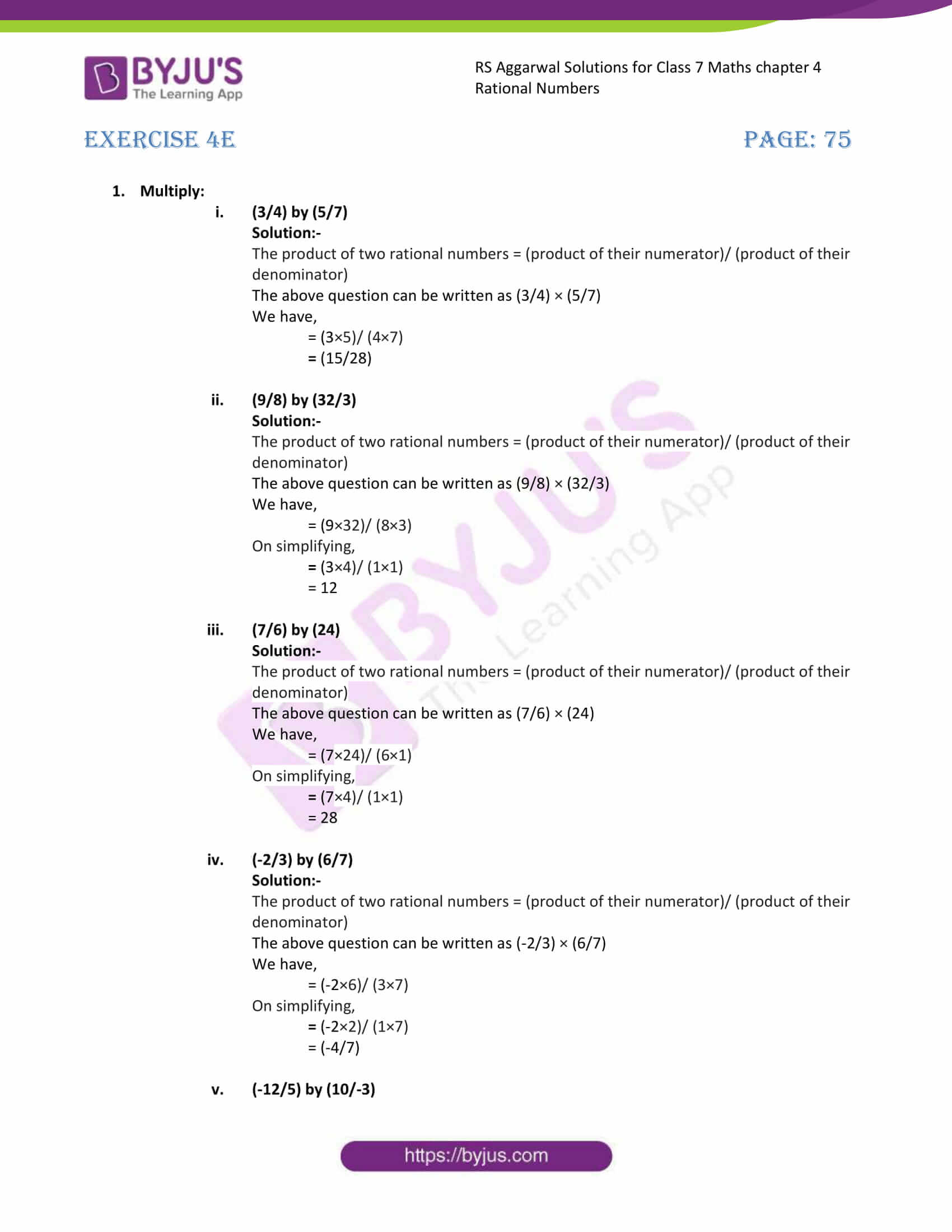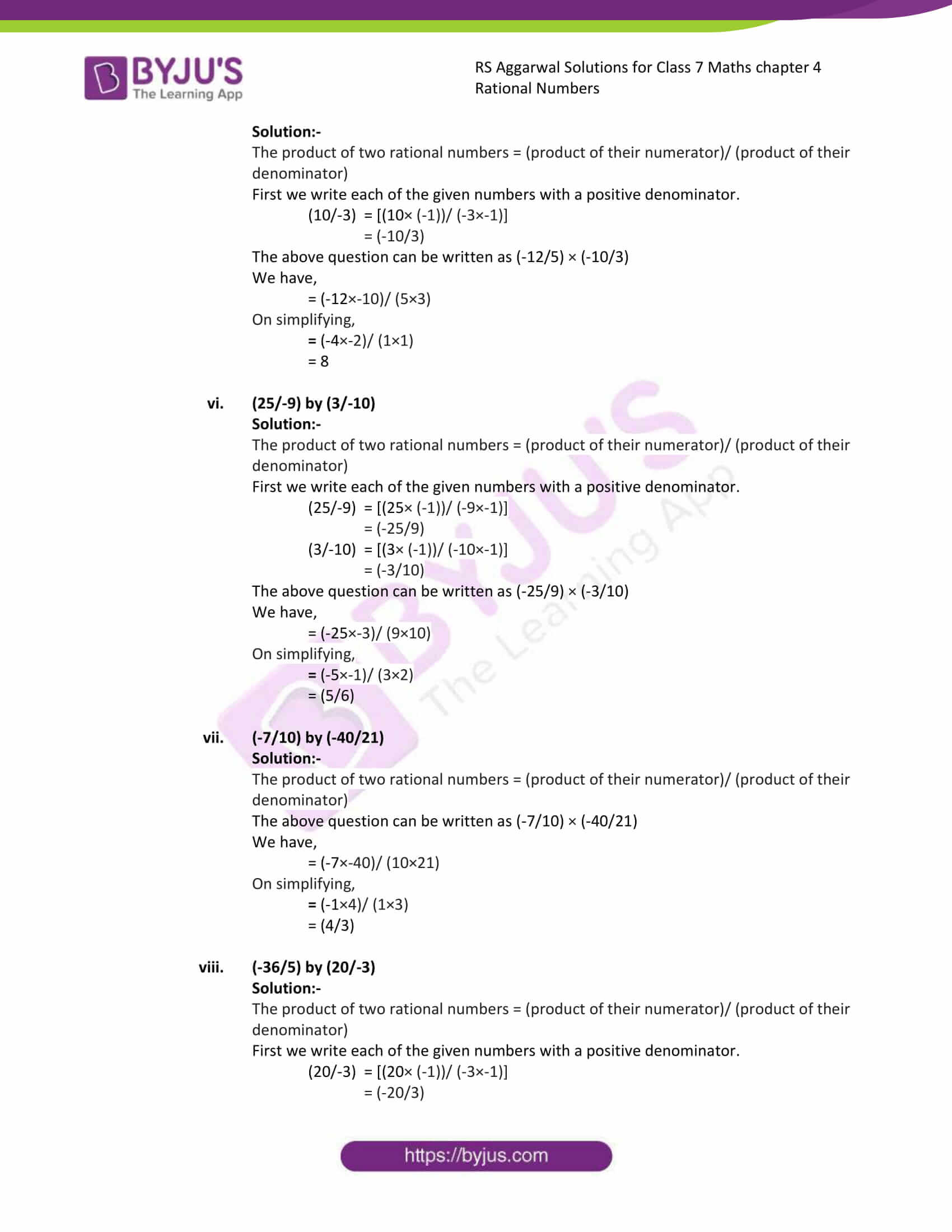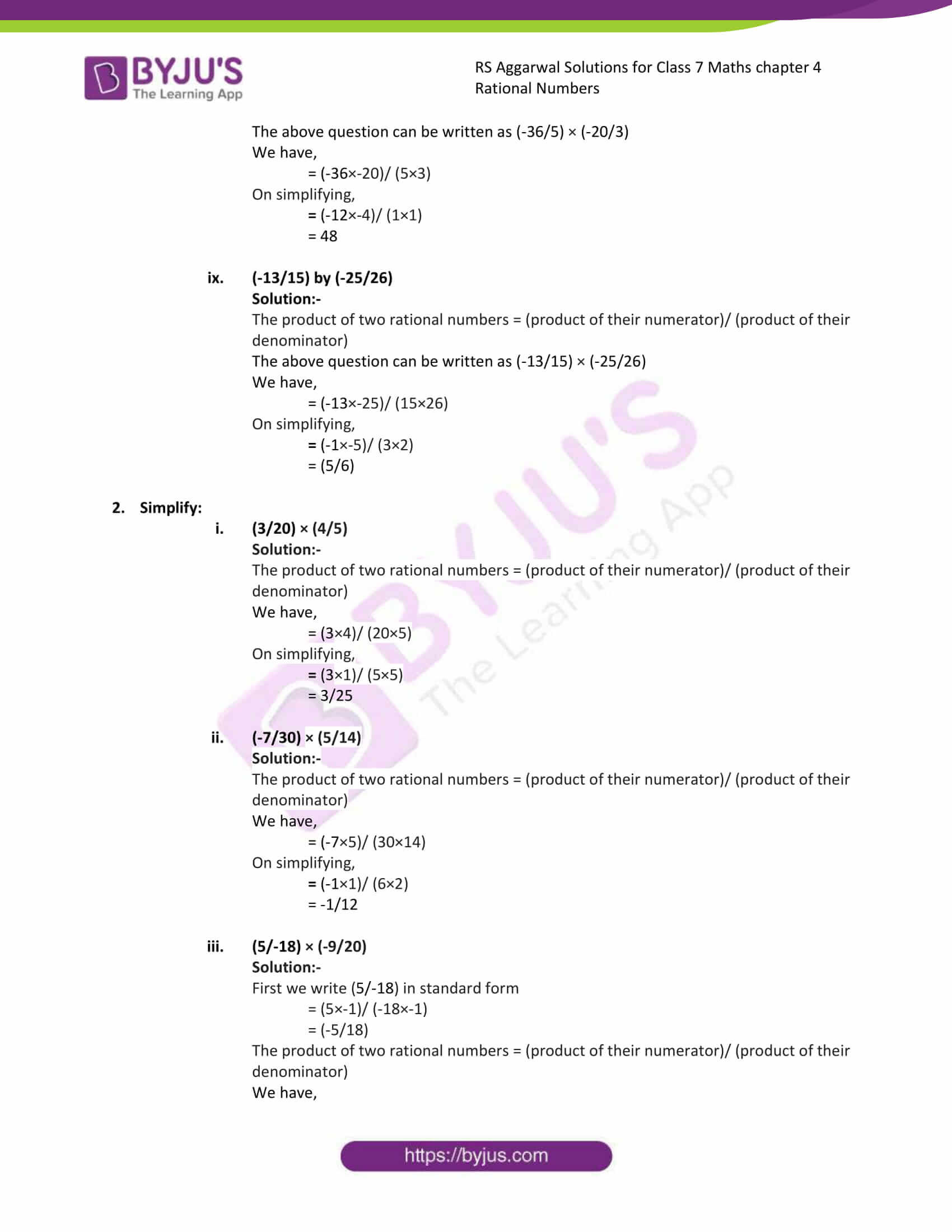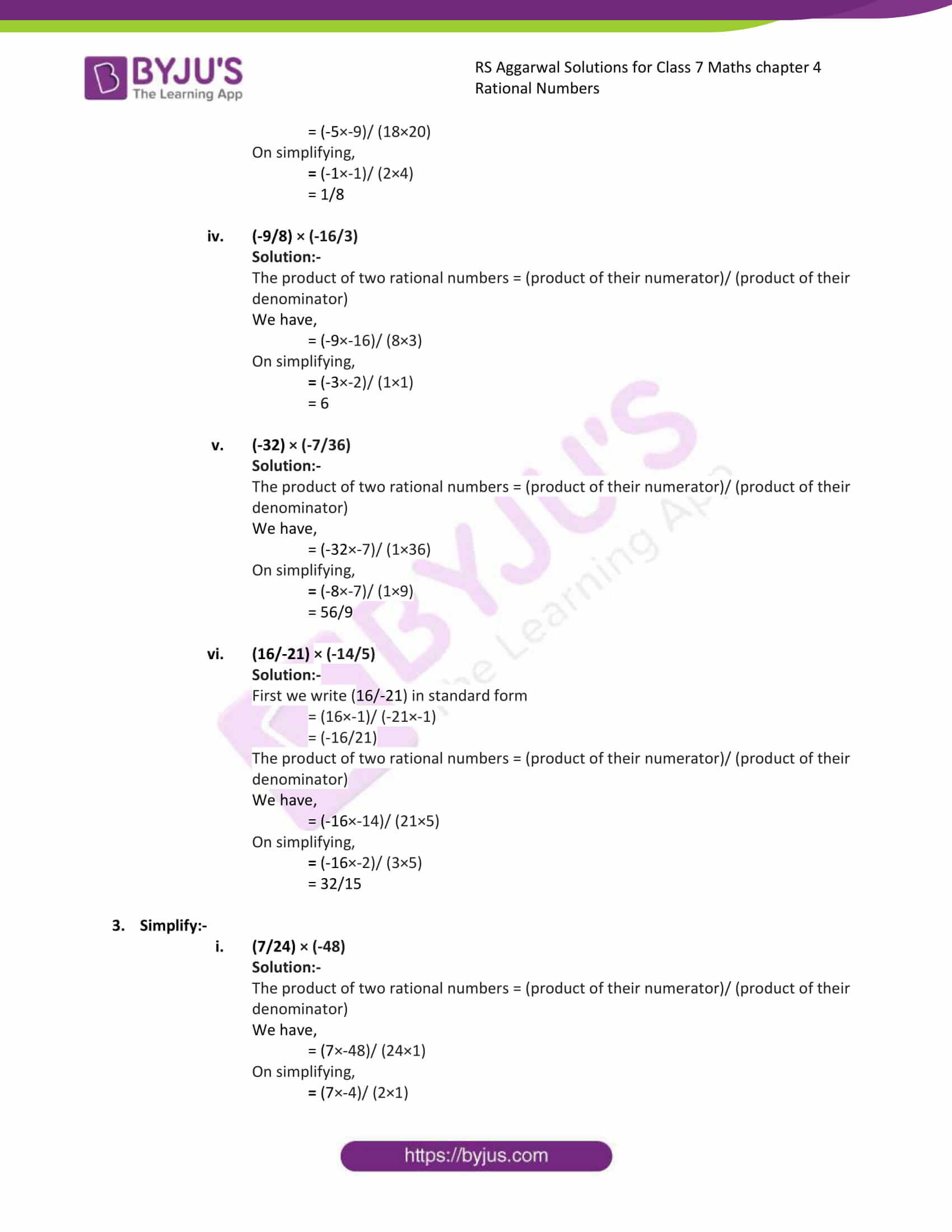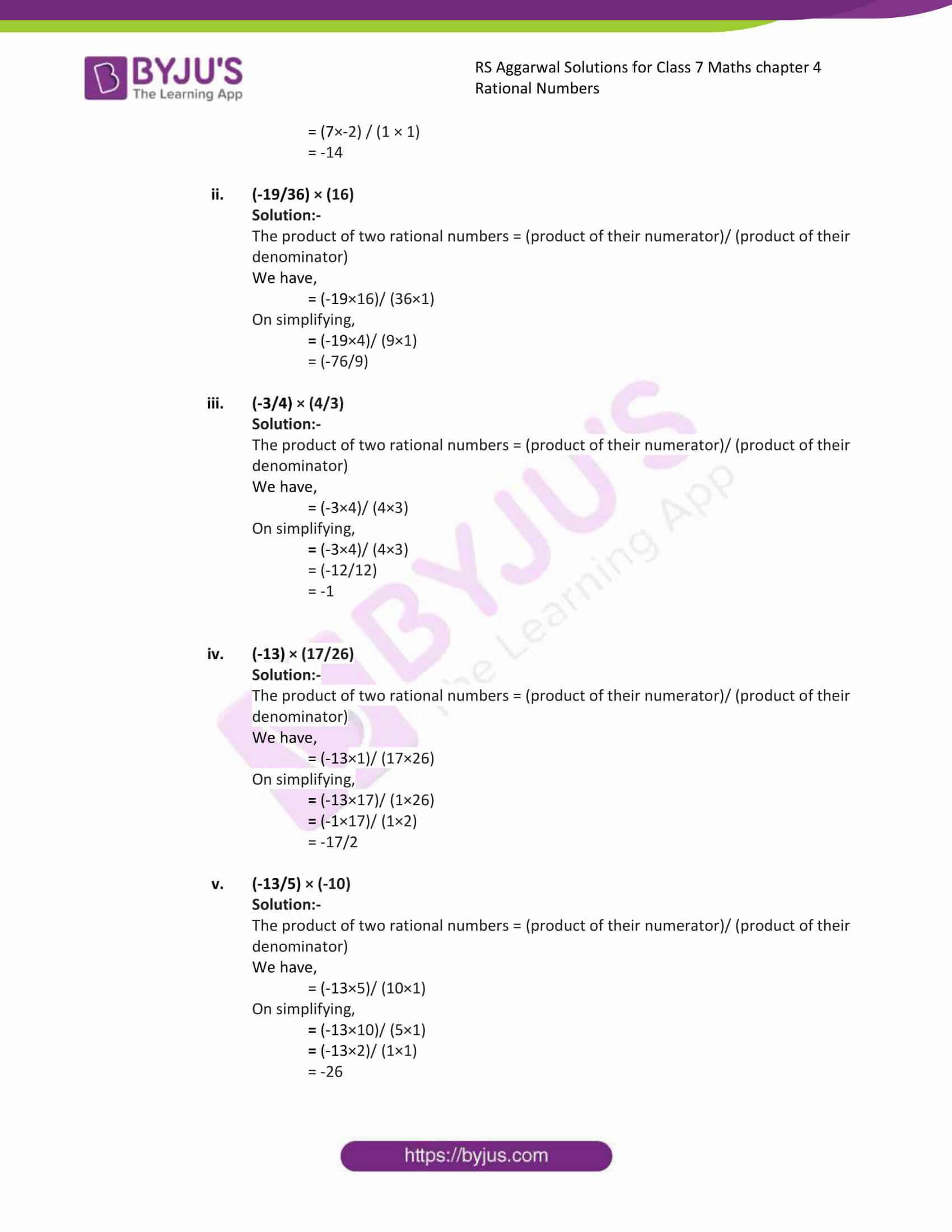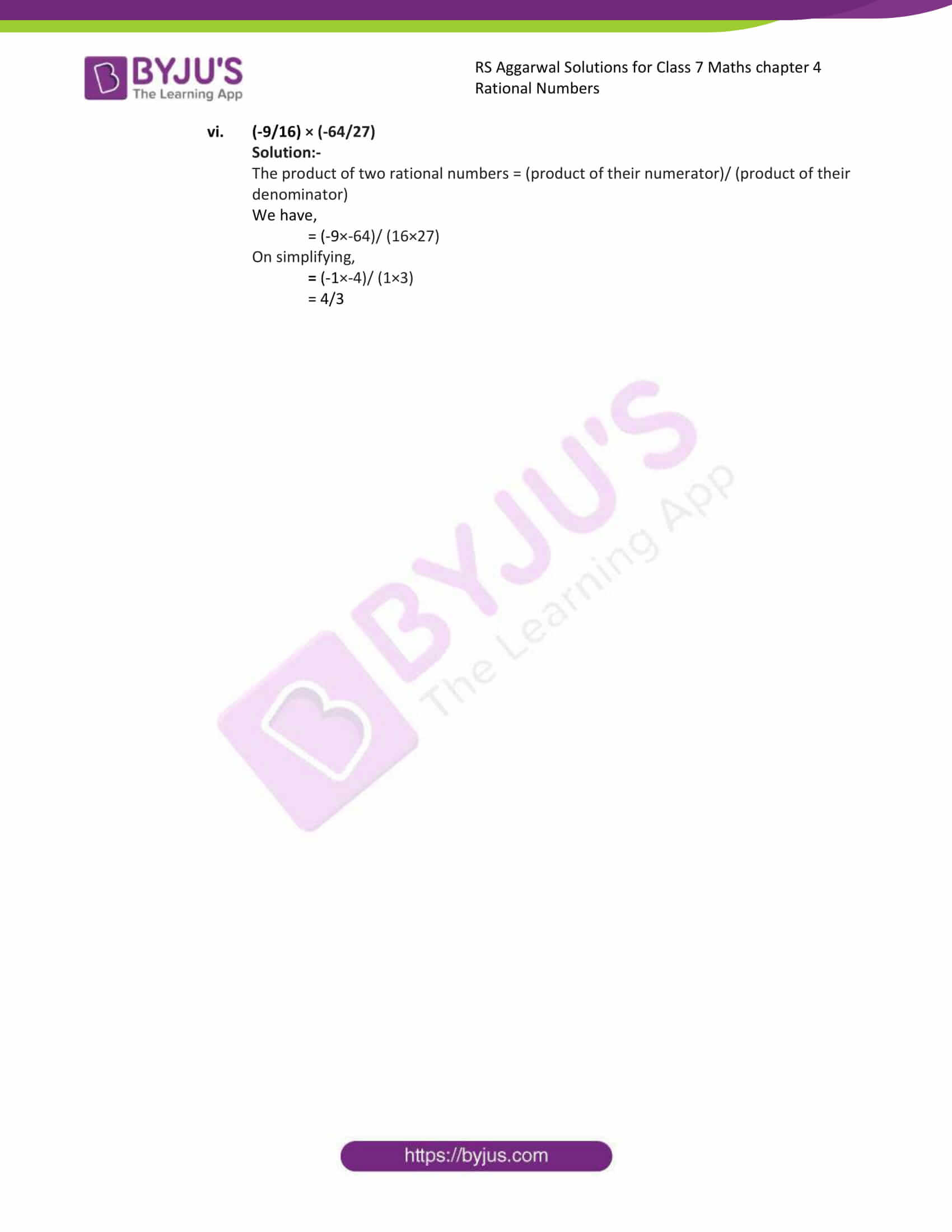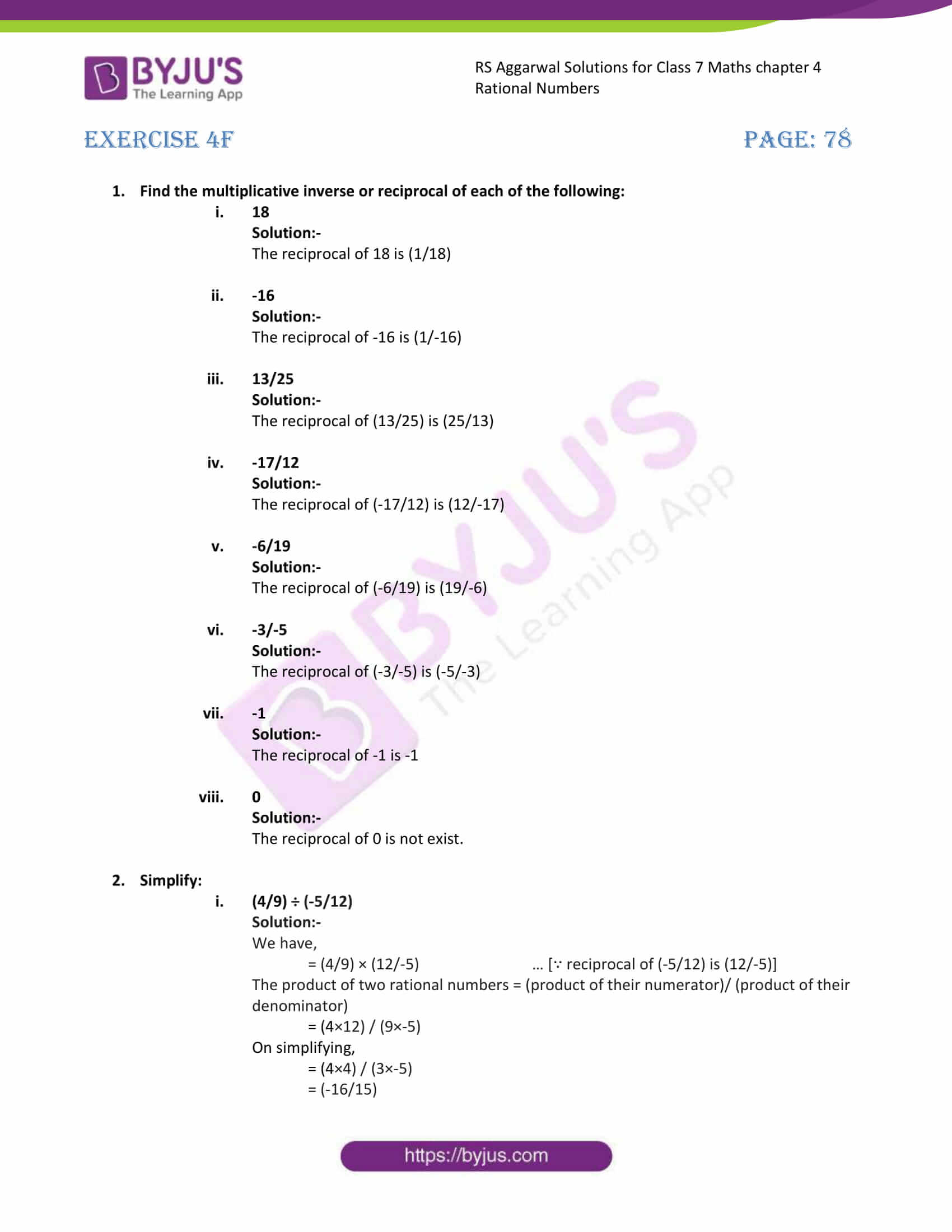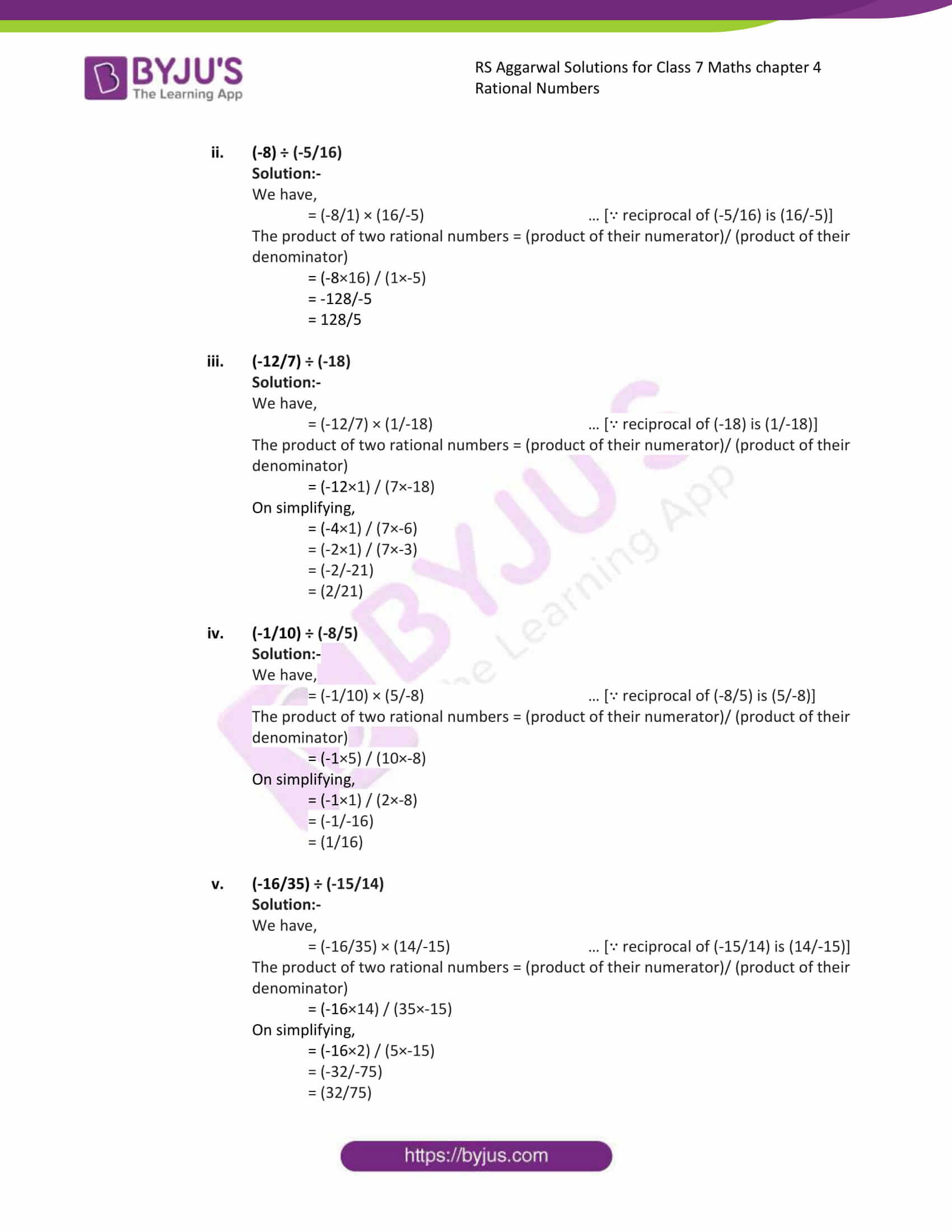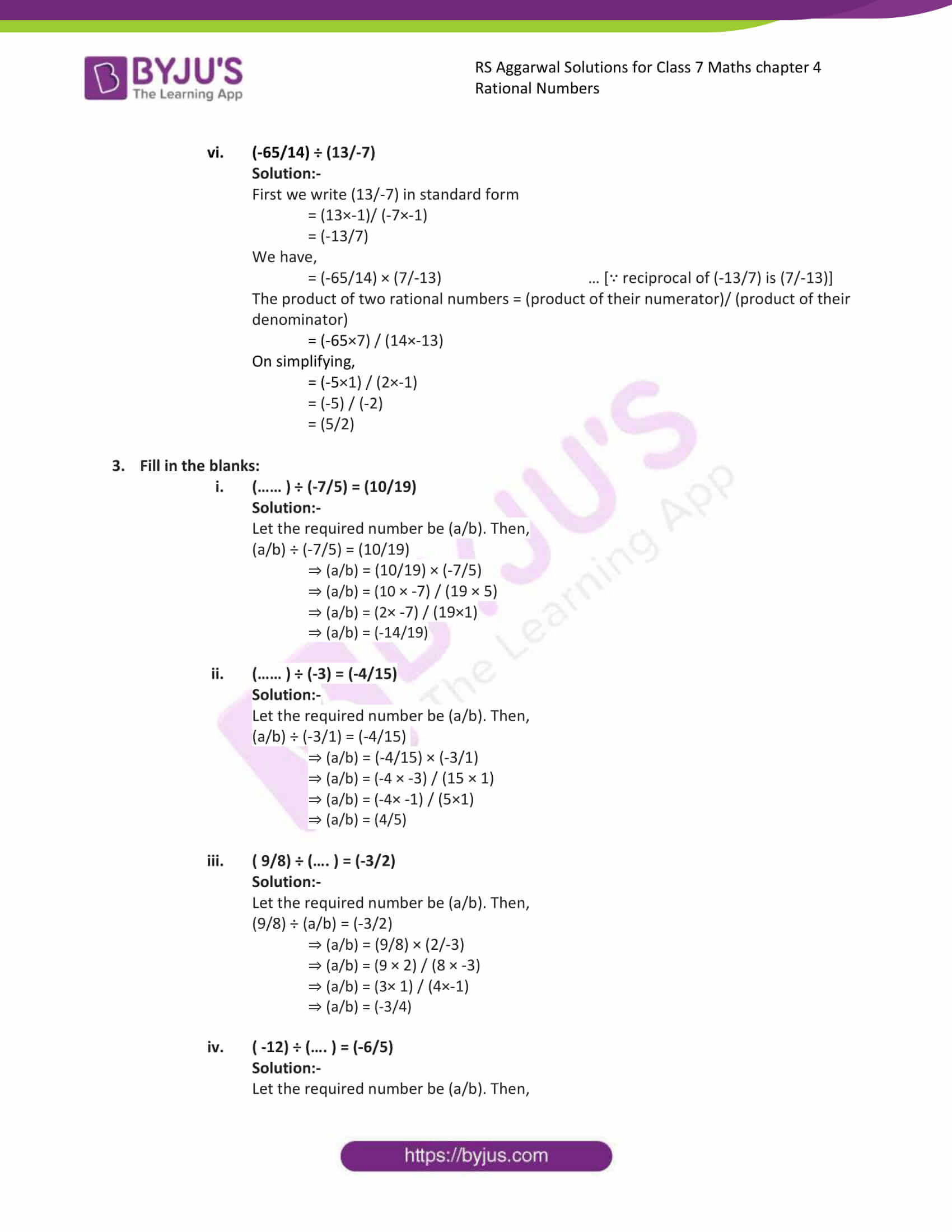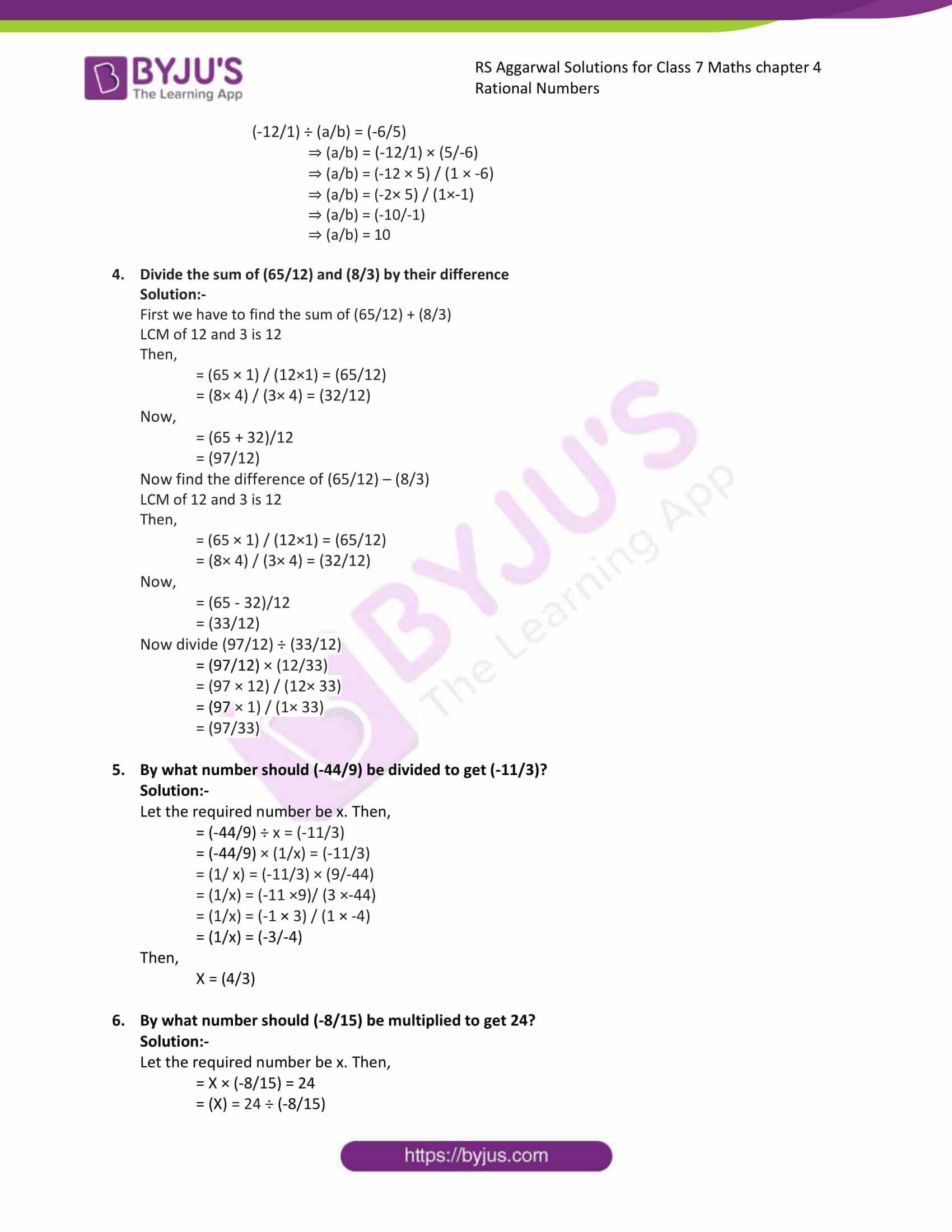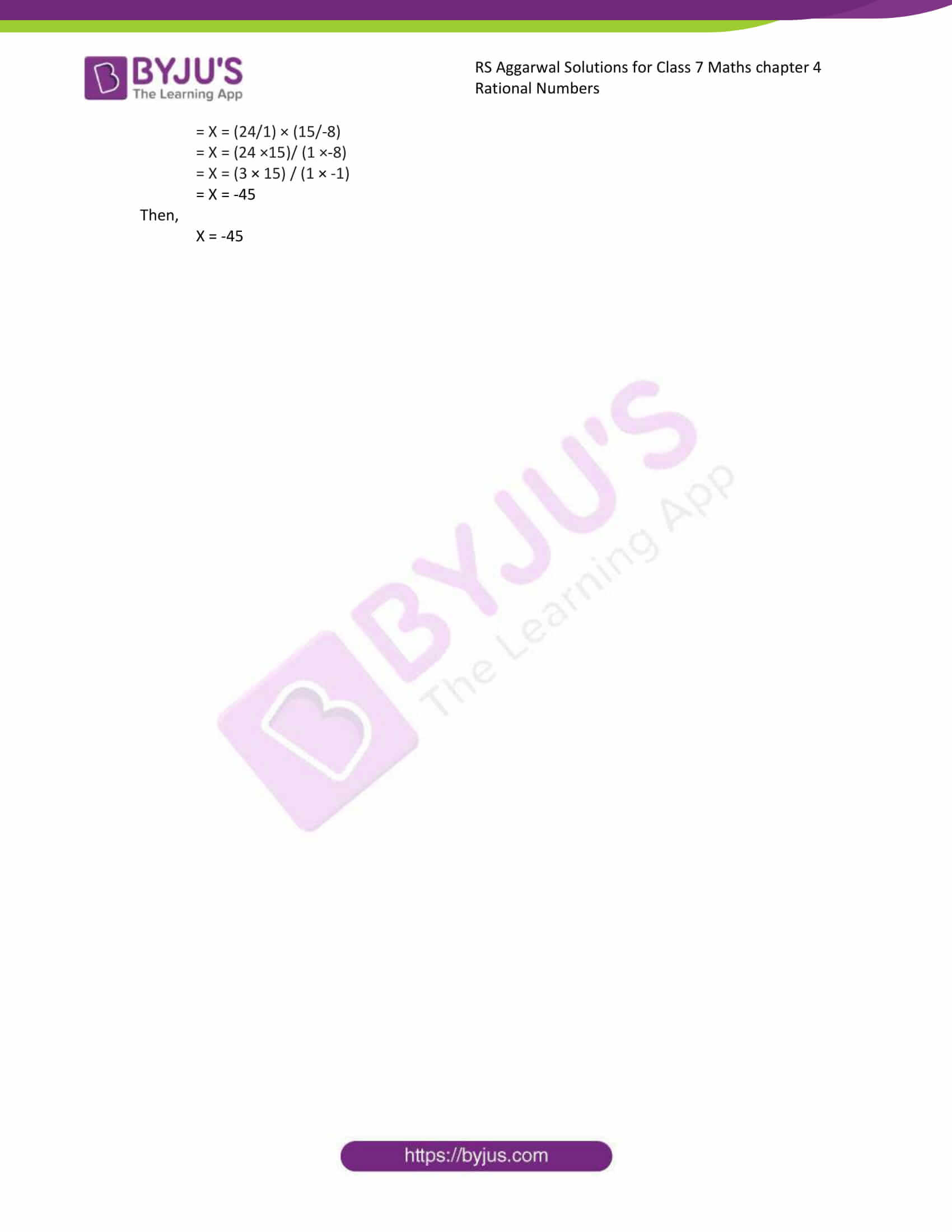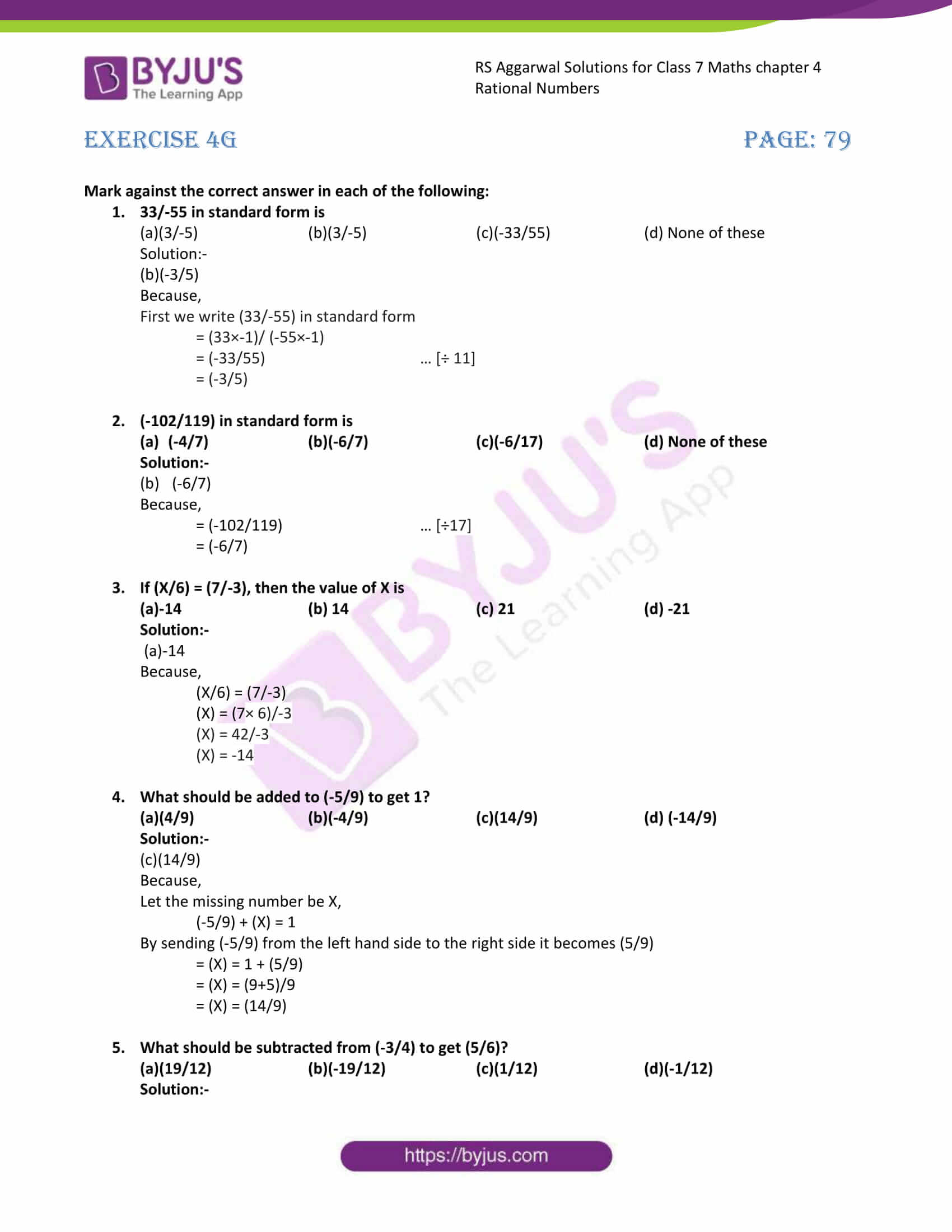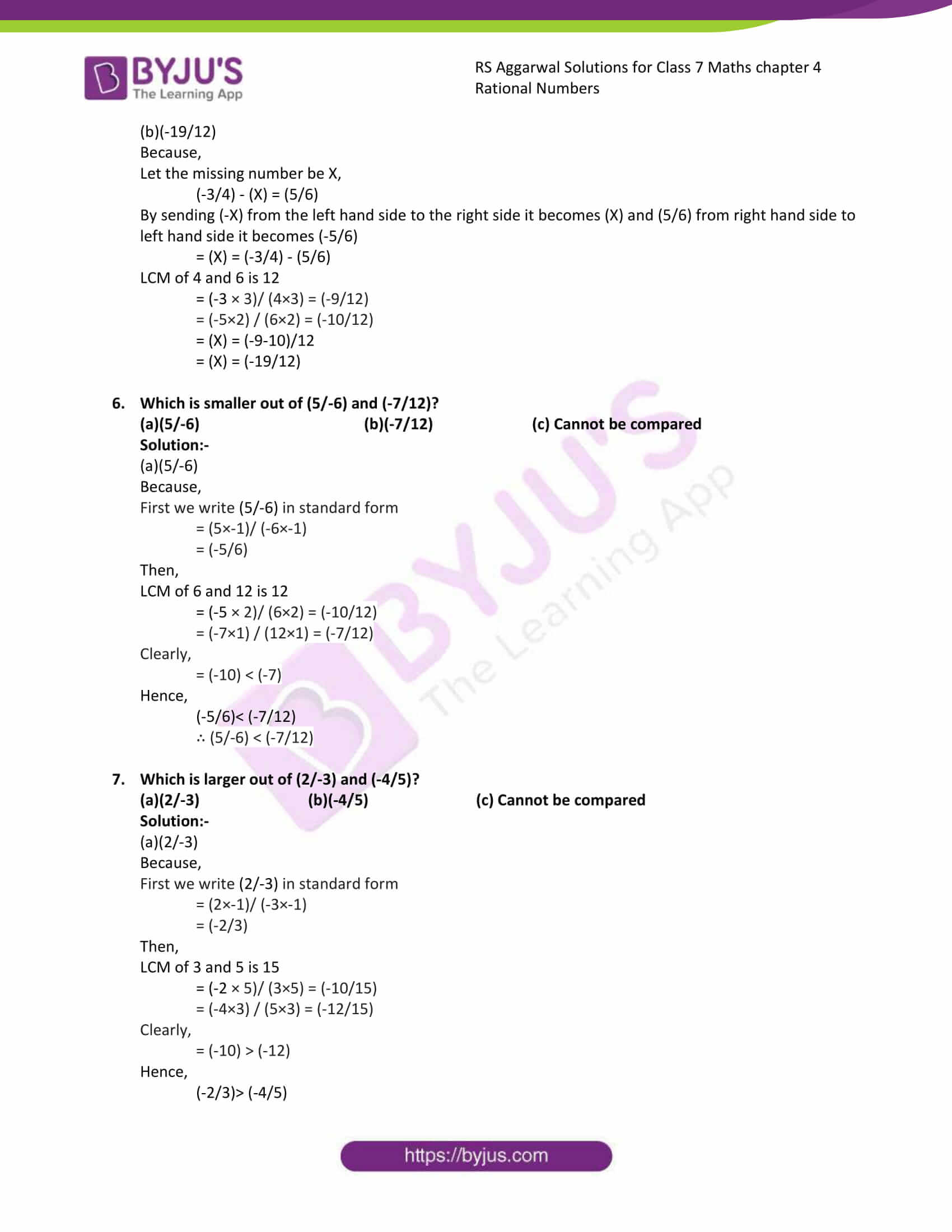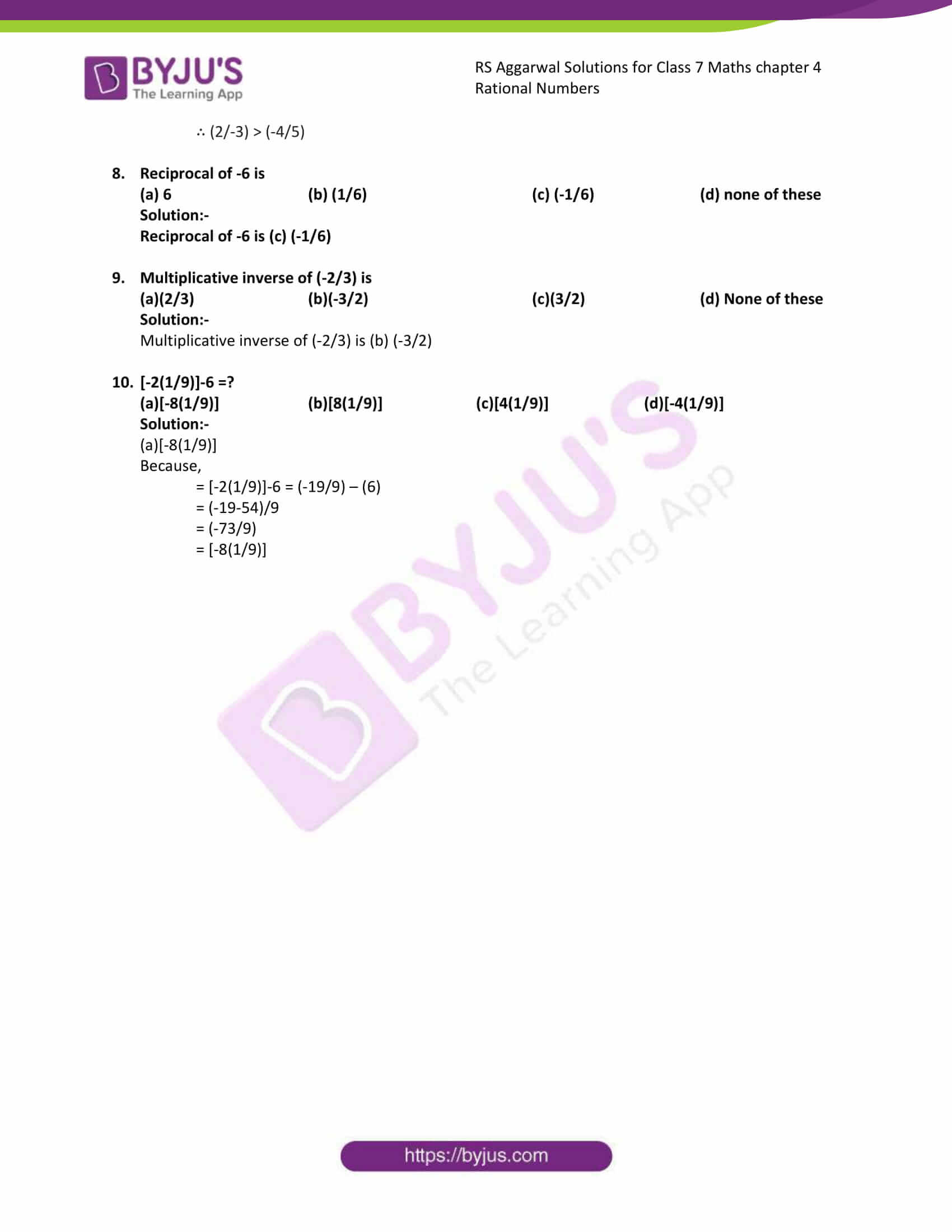Exercise 4A

Exercise 4B

Exercise 4C

Exercise 4D

Exercise 4E

Exercise 4F

Exercise 4G

## Exercise 4A

1. What are rational numbers? Give examples of five positive and five negative rational numbers. Is there any rational number which is neither positive nor negative? Name it.

The numbers of the form (p/q), where p and q are integers and q ≠ 0, are called rational numbers.

Examples for positive rational numbers: – (2/3), (-4/-5), (1/2), (20/30), (-50/-40)

Examples for negative rational numbers: – (4/-5), (-2/3), (10/-56), (46/-34), (-23/32)

Yes. There is a number which is neither positive nor negative, i.e. Zero (0)

2. Which of the following are rational numbers?

(i)(5/-8) (ii)(-6/11) (iii)(7/15) (iv)(-8/-12) (v)6 (vi)-3 (vii)0 (viii)(0/1) (ix)(1/0) (x)(0/0)

Solution:-

Rational numbers:-

(5/-8), (-6/11), (7/15), (-8/12), 6, (-3), 0, (0/1)

Because all fractions are rational number.

But, (1/0) and (0/0) are not rational number. Because these fractions have indefinite value.

3. Write down the numerator and the denominator of each of the following rational numbers:

(i)(8/19) (ii)(5/-8) (iii)(-13/15) (iv)(-8/-11) (v)(9)

Solution:-

(i) (8/19)

8 = Numerator

19= Denominator

(ii) (5/-8)

5 = Numerator

-8= Denominator

(iii) (-13/15)

-13 = Numerator

15= Denominator

(iv) (-8/-11)

-8 = Numerator

-11= Denominator

(v) 9

9 = Numerator

1= Denominator

4. Write each of the following integers as a rational number. Write the numerator and the denominator in each case.

(i)5 (ii)-3 (iii)1 (iv)0 (v)-23

Solution:-

(i) 5

Rational number= (5/1)

5 = Numerator

1= Denominator

(ii) -3

Rational number= (-3/1)

-3 = Numerator

1= Denominator

(iii) 1

Rational number= (1/1)

1 = Numerator

1= Denominator

(iv) 0

Rational number= (0/1)

0 = Numerator

1= Denominator

(v) -23

Rational number= (-23/1)

-23= Numerator

1= Denominator

5. Which of the following are positive rational numbers?

(i) (3/-5) (ii)(-11/15) (iii)(-5/-8) (iv)(37/53) (v)(0/3)

(vi)8

Solution:-

(-5/-8), (37/53), 8 these are positive rational numbers. Because numerator and denominator are either both positive or both negative.

6. Which of the following are negative rational numbers?

(i) (-15/-4) (ii)0 (iii)(-5/7) (iv)(4/-9) (v)-6

(vi)(1/-2)

Solution:-

(-5/7), (4/-9), -6, (1/-2) these are negative rational numbers. Because numerator and denominator are such that one of them is a positive integer and the other is a negative integer.

7. Find four rational numbers equivalent to each of the following.

(i)(6/11) (ii)(-3/8) (iii)(7/-15) (iv)8 (v)1

(vi)-1

Solution:-

The four rational numbers equivalent to (6/11),

= [(6×2)/ (11×2)], [(6×3)/ (11×3)], [(6×4)/ (11×4), [(6×5)/ (11×5)]

= (12/22), (18/33), (24/44), (30/55)

The four rational numbers equivalent to (-3/8),

= [(-3×2)/ (8×2)], [(-3×3)/ (8×3)], [(-3×4)/ (8×4), [(-3×5)/ (8×5)]

= (-6/16), (-9/24), (-12/32), (-15/40)

The four rational numbers equivalent to (7/-15),

= [(7×2)/ (-15×2)], [(7×3)/ (-15×3)], [(7×4)/ (-15×4), [(7×5)/ (-15×5)]

= (14/-30), (21/-45), (28/-60), (35/-75)

The four rational numbers equivalent to (8/1),

= [(8×2)/ (1×2)], [(8×3)/ (1×3)], [(8×4)/ (1×4), [(8×5)/ (1×5)]

= (16/2), (24/3), (32/4), (40/5)

The four rational numbers equivalent to (1/1),

= [(1×2)/ (1×2)], [(1×3)/ (1×3)], [(1×4)/ (1×4), [(1×5)/ (1×5)]

= (2/2), (3/3), (4/4), (5/5)

The four rational numbers equivalent to (-1/1),

= [(-1×2)/ (1×2)], [(-1×3)/ (1×3)], [(-1×4)/ (1×4), [(-1×5)/ (1×5)]

= (-2/2), (-3/3), (-4/4), (-5/5)

8. Write each of the following as a rational number with positive denominator.
(i) (12/-17)

Solution:-

The denominator of rational number is negative then we multiply its numerator and denominator by -1 to get an equivalent rational number with positive denominator.

= [(12×-1)/ (-17/-1)]

= (-12/17)

(ii) (1/-2)

Solution:-

The denominator of rational number is negative then we multiply its numerator and denominator by -1 to get an equivalent rational number with positive denominator.

= [(1×-1)/ (-2/-1)]

= (-1/2)

(iii) (-8/-19)

Solution:-

The denominator of rational number is negative then we multiply its numerator and denominator by -1 to get an equivalent rational number with positive denominator.

= [(-8×-1)/ (-19/-1)]

= (8/1)

(iv) (11/-6)

Solution:-

The denominator of rational number is negative then we multiply its numerator and denominator by -1 to get an equivalent rational number with positive denominator.

= [(11×-1)/ (-6/-1)]

= (-11/6)

9. Express (5/8) as a rational number with numerator
(i) 15

Solution:-

To get 15 in the numerator multiply by 3 for both numerator and denominator.

Then we get,

= [(5×3)/ (8×3)]

= (15/24)

(ii) -10

Solution:-

To get -10 in the numerator multiply by -2 for both numerator and denominator.

Then we get,

= [(5× (-2))/ (8× (-2))]

= (-10/-16)

10. Express (4/7) as a rational number with denominator
(i) 21

Solution:-

To get 21 in the denominator multiply by 3 for both numerator and denominator.

Then we get,

= [(4×3)/ (7×3)]

= (12/21)

(ii) -35

Solution:-

To get -35 in the denominator multiply by -5 for both numerator and denominator.

Then we get,

= [(4× (-5))/ (7× (-5))]

= (-20/-25)

11. Express (-12/13) as a rational number with numerator
(i) -48

Solution:-

To get -48 in the numerator multiply by 4 for both numerator and denominator.

Then we get,

= [(-12×4)/ (13×4)]

= (-48/52)

(ii) 60

Solution:-

To get 60 in the numerator multiply by -5 for both numerator and denominator.

Then we get,

= [(-12×-5)/ (13×-5)]

= (60/-65)

## Exercise 4B

1. Represent each of the following rational numbers on the number line: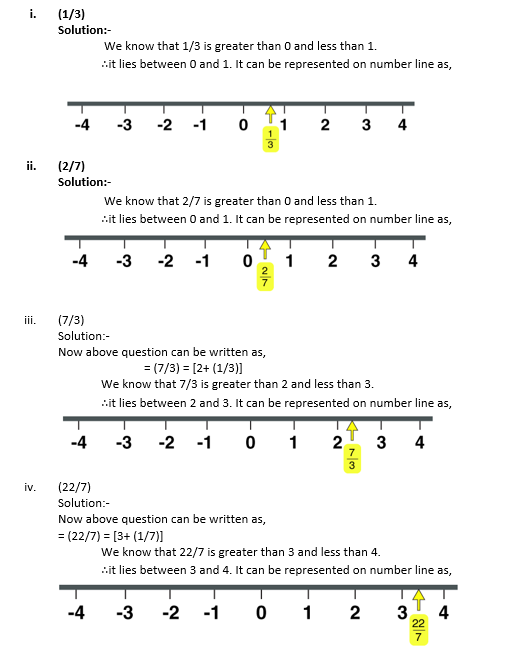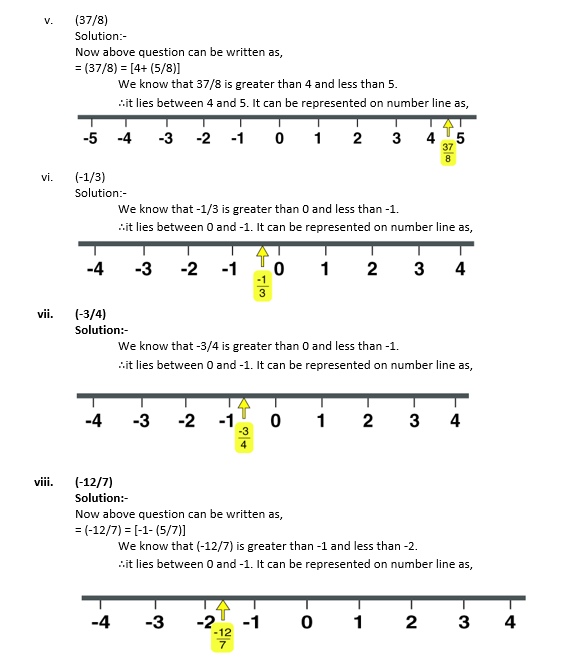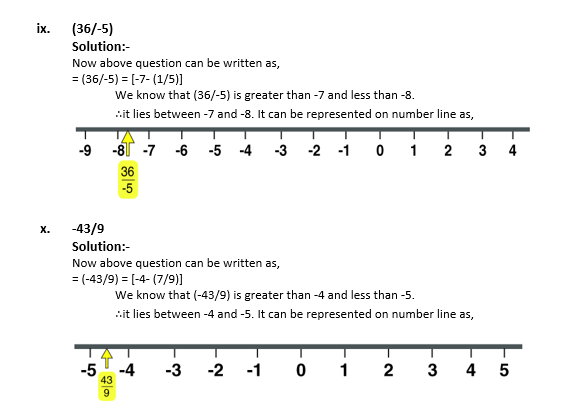2. Which of the two rational numbers is greater in each of the following pairs?
(i) (5/6) or 0

Solution:-

Since every positive rational number is greater than 0.

We have:

= (5/6) > (0)

(ii) (-3/5) or 0

Solution:-

Since every negative rational number is less than 0.

We have:

= (-3/5) < 0

(iii) (5/8) or (3/8)

Solution:-

Since both denominators are same therefore compare the numerators.

We have,

= 5 > 3

∴ (5/8) > (3/8)

(iv) (7/9) or (-5/9)

Solution:-

Since both denominators are same therefore compare the numerators.

We have,

= 7 > -5

∴ (7/9) > (-5/9)

(v) (-6/11) or (5/11)

Solution:-

Since both denominators are same therefore compare the numerators.

We have,

= -6 < 5

∴ (-6/11) < (5/11)

(vi) (-15/4) or (-17/4)

Solution:-

Since both denominators are same therefore compare the numerators.

We have,

= -15 > -17

∴ (-15/4) > (-17/4)

3. Which of the two rational number is grater in each of the following pairs?
(i) (5/9) or (-3/8)

Solution:-

The LCM of the denominators 9 and 8 is 72

∴ (5/9) = [(5×8)/ (9×8)] = (40/72)

and (-3/8) = [(-3×9)/ (8×9)] = (-27/72)

Now, 40 > -27

⇒ (40/72) > (-27/72)

Hence, (5/9) > (-3/8)

∴ 5/9 is greater.

(ii) (4/-3) or (-8/7)

Solution:-

First we write each of the given numbers with a positive denominator.

= (4/-3)

= [(4× (-1))/ (-3×-1)]

= (-4/3)

The LCM of the denominators 3 and 7 is 21

∴ (-4/3) = [(-4×7)/ (3×7)] = (-28/21)

and (-8/7) = [(-8×3)/ (7×3)] = (-24/21)

Now, -28 < -24

⇒ (-28/21) < (-24/21)

⇒ (-4/3) < (-8/7)

Hence, (4/-3) < (-8/7)

∴ -8/7 is greater.

(iii) (-12/5) or -3

Solution:-

The LCM of the denominators 5 and 1 is 5

∴ (-12/5) = [(-12×1)/ (5×1)] = (-12/5)

and (-3/1) = [(-3×5)/ (1×5)] = (-15/5)

Now, -12 > -15

⇒ (-12/5) > (-15/5)

Hence, (-12/5) > (-3)

∴ -3 is greater.

(iv) (7/-9) or (-5/8)

Solution:-

First we write each of the given numbers with a positive denominator.

= (7/-9)

= [(7× (-1))/ (-9×-1)]

= (-7/9)

The LCM of the denominators 9 and 8 is 72

∴ (-7/9) = [(-7×8)/ (9×8)] = (-56/72)

and (-5/8) = [(-5×9)/ (8×9)] = (-45/72)

Now, -56 < -45

⇒ (-56/72) < (-45/72)

⇒ (-7/9) < (-5/8)

Hence, (7/-9) < (-5/8)

∴ (-5/8) is greater.

(v) (4/-5) or (-7/8)

Solution:-

First we write each of the given numbers with a positive denominator.

= (4/-5)

= [(4× (-1))/ (-5×-1)]

= (-4/5)

The LCM of the denominators 5 and 8 is 40

∴ (-4/5) = [(-4×8)/ (5×8)] = (-32/40)

and (-7/8) = [(-7×5)/ (8×5)] = (-35/40)

Now, -32 > -35

⇒ (-32/40) > (-35/40)

⇒ (-4/5) > (-7/8)

Hence, (4/-5) > (-7/8)

∴ (4/-5) is greater.

(vi) (9/-13) or (7/-12)

Solution:-

First we write each of the given numbers with a positive denominator.

One number = (9/-13)

= [(9× (-1))/ (-13×-1)]

= (-9/13)

One number = (7/-12)

= [(7× (-1))/ (-12×-1)]

= (-7/12)

The LCM of the denominators 13 and 12 is 156

∴ (-9/13) = [(-9×12)/ (13×12)] = (-108/156)

and (-7/12) = [(-7×13)/ (12×13)] = (-91/156)

Now, -108 < -91

⇒ (-108/156) < (-91/156)

⇒ (-9/13) < (-7/12)

Hence, (9/-13) < (7/-12)

∴ (7/-12) is greater.

4. Fill in the blanks with the correct symbol out of >, < and =:
(i) (-3/7)….. (6/-13)

Solution:-

(-3/7) > (6/-13)

Because,

First we write each of the given numbers with a positive denominator.

= (6/-13)

= [(6× (-1))/ (-13×-1)]

= (-6/13)

The LCM of the denominators 7 and 13 is 91

∴ (-3/7) = [(-3×13)/ (7×13)] = (-39/91)

and (-6/13) = [(-6×7)/ (13×7)] = (-42/91)

Now, -39 > -42

⇒ (-39/91) > (-42/91)

⇒ (-3/7) > (-6/13)

Hence, (-3/7) > (6/-13)

(ii) (5/-13)…. (-35/91)

Solution:-

(5/-13)= (-35/91)

Because,

First we write each of the given numbers with a positive denominator.

= (5/-13)

= [(5× (-1))/ (-13×-1)]

= (-5/13)

The LCM of the denominators 13 and 91 is 91

∴ (-5/13) = [(-5×7)/ (13×7)] = (-35/91)

and (-35/91) = [(-35×1)/ (91×1)] = (-35/91)

Now, -35 = -35

⇒ (-35/91) = (-35/91)

⇒ (-5/13) = (-35/91)

Hence, (5/-13) = (-35/91)

(iii) -2…. (-13/5)

Solution:-

-2 > (-13/5)

Because,

The LCM of the denominators 1 and 5 is 5

∴ (-2/1) = [(-2×5)/ (1×5)] = (-10/5)

and (-13/5) = [(-13×1/ (5×1)] = (-13/5)

Now, -10 > -13

⇒ (-10/5) > (-13/5)

Hence, -2 > (-13/5)

(iv) (-2/3)…. (5/-8)

Solution:-

(-2/3) < (5/-8)

Because,

First we write each of the given numbers with a positive denominator.

= (5/-8)

= [(5× (-1))/ (-8×-1)]

= (-5/8)

The LCM of the denominators 3 and 8 is 24

∴ (-2/3) = [(-2×8)/ (3×8)] = (-16/24)

and (-5/8) = [(-5×3)/ (8×3)] = (-15/24)

Now, -16 = -15

⇒ (-16/24) < (-15/24)

⇒ (-2/3) < (-5/8)

Hence, (-2/3) < (5/-8)

(v) 0….. (-3/-15)

Solution:-

0 < (-3/-15)

Because,

First we write each of the given numbers with a positive denominator.

= (-3/-15)

= [(-3× (-1))/ (-15×-1)]

= (3/15)

The LCM of the denominators 1 and 15 is 15

∴ (0/1) = [(0×15)/ (1×15)] = (0/15)

and (3/15) = [(3×1)/ (15×1)] = (3/15)

Now, 0 < 3

⇒ (0/15) < (3/15)

⇒ 0 < (3/15)

Hence, 0 < (-3/-15)

(vi) (-8/9)…… (-9/10)

Solution:-

(-8/9) > (-9/10)

The LCM of the denominators 9 and 10 is 90

∴ (-8/9) = [(-8×10)/ (9×10)] = (-80/90)

and (-9/10) = [(-9×9)/ (10×9)] = (-81/90)

Now, -80 > -81

⇒ (-80/90) > (-81/90)

Hence, (-8/9) > (-9/10)

5. Arrange the following rational numbers in ascending order:
(i) (2/5), (7/10), (8/15), (13/30)

Solution:-

LCM of 5, 10, 15 and 30 is 30

Now,

(2/5) = [(2×6)/ (5×6)] = (12/30)

(7/10) = [(7×3)/ (10×3)] = (21/30)

(8/15) = [(8×2)/ (15×2)] = (16/30)

(13/30) = [(13×1)/ (30×1)] = (13/30)

Clearly,

(12/30) < (13/30) < (16/30) < (21/30)

Hence,

(2/5) < (13/30) < (16/30) < (21/30)

(ii) (-3/4), (5/-12), (-7/16), (9/-24)

Solution:-

First we write each of the given numbers with a positive denominator.

(5/-12) = [(5× (-1))/ (-12×-1)]

= (-5/12)

(9/-24) = [(9× (-1))/ (-24×-1)]

= (-9/24)

LCM of 4, 12, 16 and 24 is 48

Now,

(-3/4)= [(-3×12)/ (4×12)] = (-36/48)

(-5/12)= [(-5×4)/ (12×4)] = (-20/48)

(-7/16)= [(-7×3)/ (16×3)] = (-21/48)

(-9/24)= [(-9×2)/ (24×2)] = (-18/48)

Clearly,

(-36/48)< (-21/48) < (-20/48) < (-18/48)

Hence,

(-3/4)< (-7/16) < (5/-12) < (9/-24)

(iii) (-3/10), (7/-15), (-11/20), (17/-30)

Solution:-

First we write each of the given numbers with a positive denominator.

(7/-15) = [(7× (-1))/ (-15×-1)]

= (-7/15)

(17/-30)= [(17× (-1))/ (-30×-1)]

= (-17/30)

LCM of 10, 15, 20 and 30 is 60

Now,

(-3/10)= [(-3×6)/ (10×6)] = (-18/60)

(-7/15)= [(-7×4)/ (15×4)] = (-28/60)

(-11/20)= [(-11×3)/ (20×3)] = (-33/60)

(-17/30)= [(-13×2)/ (30×2)] = (-26/60)

Clearly,

(-33/60)< (-28/60) < (-26/60) < (-18/60)

Hence,

(-11/20)< (-7/15) < (-17/30) < (-3/10)

(iv) (2/3), (3/4), (5/-6), (-7/12)

Solution:-

First we write each of the given numbers with a positive denominator.

(5/-6) = [(5× (-1))/ (-6×-1)]

= (-5/6)

LCM of 3, 4, 6 and 12 is 12

Now,

(2/3)= [(2×4)/ (3×4)] = (8/12)

(3/4)= [(3×3)/ (4×3)] = (9/12)

(-5/6)= [(-5×2)/ (6×2)] = (-10/12)

(-7/12)= [(-7×1)/ (12×1)] = (-7/12)

Clearly,

(-10/12)< (-7/12) < (8/12) < (9/12)

Hence,

(-5/6)< (-7/15) < (2/3) < (3/4)

## Exercise 4C

1. Add the following rational numbers :
(i) (12/7) and (3/7)

Solution:-

We have:

= [(12 + 3)/7] … [∵ denominator is same in both the rational numbers]

= (15/7)

(ii) (-2/5) and (1/5)

Solution:-

We have:

= [(-2 + 1)/5] … [∵ denominator is same in both the rational numbers]

= (-1/5)

(iii) (3/-8) and (1/8)

Solution:-

First we write each of the given numbers with a positive denominator.

(3/-8) = [(3× (-1))/ (-8×-1)]

= (-3/8)

Then,

(-3/8)+ (1/8)

We have:

= [(-3 + 1)/8] … [∵ denominator is same in both the rational numbers]

= (-2/8)

(iv) (-5/11) and (7/-11)

Solution:-

First we write each of the given numbers with a positive denominator.

(7/-11) = [(7× (-1))/ (-11×-1)]

= (-7/11)

Then,

(-5/11)+ (-7/11)

We have:

= [(-5 + (-7))/11] … [∵ denominator is same in both the rational numbers]

= [(-5-7)/11)

= (-12/11)

(v) (9/-13) and (-11/-13)

Solution:-

First we write each of the given numbers with a positive denominator.

(9/-13) = [(9× (-1))/ (-13×-1)]

= (-9/13)

(-11/-13) = [(-11× (-1))/ (-13×-1)]

= (11/13)

Then,

(-9/13)+ (11/13)

We have:

= [(-9 + 11)/13] … [∵ denominator is same in both the rational numbers]

= (2/13)

(vi) (-2/9) and (-5/9)

Solution:-

Then,

(-2/9)+ (-5/9)

We have:

= [(-2 + (-5)/9] … [∵ denominator is same in both the rational numbers]

= ((-2-5)/9)

= (-7/9)

(vii) (-17/9) and (-11/9)

Solution:-

Then,

(-17/9)+ (-11/9)

We have:

= [(-17 + (-11)/9] … [∵ denominator is same in both the rational numbers]

= ((-17-11)/9)

= (-28/9)

(viii) (-3/7) and (5/-7)

Solution:-

First we write each of the given numbers with a positive denominator.

(5/-7) = [(5× (-1))/ (-7×-1)]

= (-5/7)

Then,

(-3/7)+ (-5/7)

We have:

= [(-3 + (-5))/7] … [∵ denominator is same in both the rational numbers]

= ((-3-5)/7)

= (-8/7)

2. Add the following rational numbers:
(i) (-2/5) and (3/4)

Solution:-

Take the LCM of the denominators of the given rational numbers.

LCM of 5 and 4 is 20

Express each of the given rational numbers with the above LCM as the common denominator.

Now,

(-2/5)= [(-2×4)/ (5×4)] = (-8/20)

(3/4)= [(3×5)/ (4×5)] = (15/20)

Then,

= (-8/20) + (15/20) … [∵ denominator is same in both the rational numbers]

= (-8 + 15)/20

= (7/20)

(ii) (-5/9) and (2/3)

Solution:-

Take the LCM of the denominators of the given rational numbers.

LCM of 9 and 3 is 9

Express each of the given rational numbers with the above LCM as the common denominator.

Now,

(-5/9)= [(-5×1)/ (9×1)] = (-5/9)

(2/3)= [(2×3)/ (3×3)] = (6/9)

Then,

= (-5/9) + (6/9) … [∵ denominator is same in both the rational numbers]

= (-5 + 6)/9

= (1/9)

(iii) -4 and (1/2)

Solution:-

Take the LCM of the denominators of the given rational numbers.

LCM of 1 and 2 is 2

Express each of the given rational numbers with the above LCM as the common denominator.

Now,

(-4/1)= [(-4×2)/ (1×2)] = (-8/2)

(1/2)= [(1×1)/ (2×1)] = (1/2)

Then,

= (-8/2) + (1/2) … [∵ denominator is same in both the rational numbers]

= (-8 + 1)/2

= (-7/2)

(iv) (-7/27) and (5/18)

Solution:-

Take the LCM of the denominators of the given rational numbers.

LCM of 27 and 18 is 54

Express each of the given rational numbers with the above LCM as the common denominator.

Now,

(-7/27)= [(-7×2)/ (27×2)] = (-14/54)

(5/18)= [(5×3)/ (18×3)] = (15/54)

Then,

= (-14/54) + (15/54) … [∵ denominator is same in both the rational numbers]

= (-14 + 15)/54

= (1/54)

(v) (-5/36) and (-7/12)

Solution:-

Take the LCM of the denominators of the given rational numbers.

LCM of 36 and 12 is 36

Express each of the given rational numbers with the above LCM as the common denominator.

Now,

(-5/36)= [(-5×1)/ (36×1)] = (-5/36)

(-7/12)= [(-7×3)/ (12×3)] = (-21/36)

Then,

= (-5/36) + (-21/36) … [∵ denominator is same in both the rational numbers]

= (-5+ (-21))/36

= (-5 – 21)/36

= (-26/36)

(vi) (1/-9) and (4/-27)

Solution:-

First we write each of the given numbers with a positive denominator.

(1/-9) = [(1× (-1))/ (-9×-1)]

= (-1/9)

(4/-27) = [(4× (-1))/ (-27×-1)]

= (-4/27)

Take the LCM of the denominators of the given rational numbers.

LCM of 9 and 27 is 27

Express each of the given rational numbers with the above LCM as the common denominator.

Now,

(-1/9)= [(-1×3)/ (9×3)] = (-3/27)

(-4/27)= [(-4×1)/ (27×1)] = (-4/27)

Then,

= (-3/27) + (-4/27) … [∵ denominator is same in both the rational numbers]

= (-3 + (-4))/27

= (-3 – 4)/ 27

= (-7 / 27)

(vii) (-9/24) and (-1/18)

Solution:-

Take the LCM of the denominators of the given rational numbers.

LCM of 24 and 18 is 72

Express each of the given rational numbers with the above LCM as the common denominator.

Now,

(-9/24)= [(-9×3)/ (24×3)] = (-27/72)

(-1/18)= [(-1×4)/ (18×4)] = (-4/72)

Then,

= (-27/72) + (-4/72) … [∵ denominator is same in both the rational numbers]

= (-27+ (-4))/72

= (-27 – 4)/72

= (-31/72)

(viii) (27/-4) and (-15/8)

Solution:-

First we write each of the given numbers with a positive denominator.

(27/-4) = [(27× (-1))/ (-4×-1)]

= (-27/4)

Take the LCM of the denominators of the given rational numbers.

LCM of 4 and 8 is 8

Express each of the given rational numbers with the above LCM as the common denominator.

Now,

(-27/4)= [(-27×2)/ (4×2)] = (-54/8)

(-15/8)= [(-15×1)/ (8×1)] = (-15/8)

Then,

= (-54/8) + (-15/8) … [∵ denominator is same in both the rational numbers]

= (-54 + (-15))/8

= (-54 – 15)/ 8

= (-69 / 8)

## Exercise 4D

1. Find the additive inverse of:
(i) 5

Solution:-

Additive inverse of 5 is -5

(ii) -9

Solution:-

Additive inverse of -9 is 9

(iii) (3/14)

Solution:-

Additive inverse of (3/14) is (-3/14)

(iv) (-11/15)

Solution:-

Additive inverse of (-11/15) is (11/15)

(v) (15/-4)

Solution:-

First we write each of the given numbers with a positive denominator.

(15/-4) = [(15× (-1))/ (-4×-1)]

= (-15/4)

Then,

Additive inverse of (-15/4) is (15/4)

(vi) (-18/-13)

Solution:-

First we write each of the given numbers with a positive denominator.

(-18/-13) = [(-18× (-1))/ (-13×-1)]

= (18/13)

Then,

Additive inverse of (18/13) is (-18/13)

(vii) O

Solution:-

Additive inverse of 0 is 0

(viii) (1/-6)

Solution:-

First we write each of the given numbers with a positive denominator.

(1/-6) = [(1× (-1))/ (-6×-1)]

= (-1/6)

Then,

Additive inverse of (-1/6) is (1/6)

2. Subtract :
(i) (3/4) from (1/3)

Solution:-

We have:

= (1/3) – (3/4)

= (1/3) + (additive inverse of 3/4)

= (1/3) + (-3/4)

LCM of 4 and 3 is 12

Express each of the given rational numbers with the above LCM as the common denominator.

Now,

= [(1×4)/ (3×4)] = (4/12)

= [(-3×3)/ (4×3)] = (-9/12)

Then,

= (4/12) + (-9/12)

= (4-9)/12

= (-5/12)

(ii) (-5/6) from (1/3)

Solution:-

We have:

= (1/3) – (-5/6)

= (1/3) + (additive inverse of -5/6)

= (1/3) + (5/6)

LCM of 3 and 6 is 6

Express each of the given rational numbers with the above LCM as the common denominator.

Now,

= [(1×2)/ (3×2)] = (2/6)

= [(5×1)/ (6×1)] = (5/6)

Then,

= (2/16) + (5/6)

= (2+5)/6

= (7/6)

(iii) (-8/9) from (-3/5)

Solution:-

We have:

= (-3/5) – (-8/9)

= (-3/5) + (additive inverse of -8/9)

= (-3/5) + (8/9)

LCM of 5 and 9 is 45

Express each of the given rational numbers with the above LCM as the common denominator.

Now,

= [(-3×9)/ (5×9)] = (-27/45)

= [(8×5)/ (9×5)] = (40/45)

Then,

= (-27/45) + (40/45)

= (-27+40)/45

= (13/45)

(iv) (-9/7) from (-1)

Solution:-

We have:

= (-1/1) – (-9/7)

= (-1/1) + (additive inverse of -9/7)

= (-1/1) + (9/7)

LCM of 1 and 7 is 7

Express each of the given rational numbers with the above LCM as the common denominator.

Now,

= [(-1×7)/ (1×7)] = (-7/7)

= [(9×1)/ (7×1)] = (9/7)

Then,

= (-7/7) + (9/7)

= (-7+9)/7

= (2/7)

(v) (-18/11) from (1)

Solution:-

We have:

= (1/1) – (-18/11)

= (1/1) + (additive inverse of -18/11)

= (1/1) + (18/11)

LCM of 1 and 11 is 11

Express each of the given rational numbers with the above LCM as the common denominator.

Now,

= [(1×11)/ (1×11)] = (11/11)

= [(18×1)/ (11×1)] = (18/11)

Then,

= (11/11) + (18/11)

= (11+18)/11

= (29/11)

(vi) (-13/9) from (0)

Solution:-

We have:

= (-13/9) – (0)

= (0/1) + (additive inverse of -13/9)

= (0/1) + (13/9)

LCM of 1 and 9 is 9

Express each of the given rational numbers with the above LCM as the common denominator.

Now,

= [(0×9)/ (1×9)] = (0/9)

= [(13×1)/ (9×1)] = (13/9)

Then,

= (0/9) + (13/9)

= (0+13)/9

= (13/9)

(vii) (-32/13) from (-6/5)

Solution:-

We have:

= (-6/5) – (-32/13)

= (-6/5) + (additive inverse of -32/13)

= (-6/5) + (32/13)

LCM of 5 and 13 is 65

Express each of the given rational numbers with the above LCM as the common denominator.

Now,

= [(-6×13)/ (5×13)] = (-78/65)

= [(32×5)/ (13×5)] = (160/65)

Then,

= (-78/65) + (160/65)

= (-78+160)/65

= (82/65)

(viii) (-7) from (-4/7)

Solution:-

We have:

= (-4/7) – (-7/1)

= (-4/7) + (additive inverse of -7/1)

= (-4/7) + (7/1)

LCM of 7 and 1 is 7

Express each of the given rational numbers with the above LCM as the common denominator.

Now,

= [(-4×1)/ (7×1)] = (-4/7)

= [(7×7)/ (1×7)] = (49/7)

Then,

= (-4/7) + (49/7)

= (-4+49)/7

= (45/7)

(ix) (5/9) from (-2/3)

Solution:-

We have:

= (-2/3) – (5/9)

= (-2/3) + (additive inverse of 5/9)

= (-2/3) + (-5/9)

LCM of 3 and 9 is 9

Express each of the given rational numbers with the above LCM as the common denominator.

Now,

= [(-2×3)/ (3×3)] = (-6/9)

= [(-5×1)/ (9×1)] = (-5/9)

Then,

= (-6/9) + (-5/9)

= (-6-5)/9

= (-11/9)

(x) (5) from (-3/5)

Solution:-

We have:

= (5/1) – (-3/5)

= (5/1) + (additive inverse of -3/5)

= (5/1) + (3/5)

LCM of 1 and 5 is 5

Express each of the given rational numbers with the above LCM as the common denominator.

Now,

= [(5×5)/ (1×5)] = (25/5)

= [(3×1)/ (5×1)] = (3/5)

Then,

= (25/5) + (3/5)

= (25+3)/5

= (28/5)

3. Evaluate:
(i) (3/4) – (4/5)

Solution:-

We have:

= (3/4) – (4/5)

= (3/4) + (additive inverse of 4/5)

= (3/4) + (-4/5)

LCM of 4 and 5 is 20

Express each of the given rational numbers with the above LCM as the common denominator.

Now,

= [(3×5)/ (4×5)] = (15/20)

= [(-4×4)/ (5×4)] = (-16/20)

Then,

= (15/20) + (-16/20)

= (15-16)/20

= (-1/20)

(ii) (-3) – (4/7)

Solution:-

We have:

= (-3/1) – (4/7)

= (-3/1) + (additive inverse of 4/7)

= (-3/1) + (-4/7)

LCM of 1 and 7 is 7

Express each of the given rational numbers with the above LCM as the common denominator.

Now,

= [(-3×7)/ (1×7)] = (-21/7)

= [(-4×1)/ (7×1)] = (-4/7)

Then,

= (-21/7) + (-4/7)

= (-21-4)/7

= (-25/7)

(iii) (7/24) – (19/36)

Solution:-

We have:

= (7/24) – (19/36)

= (7/24) + (additive inverse of 19/36)

= (7/24) + (-19/36)

LCM of 24 and 36 is 72

Express each of the given rational numbers with the above LCM as the common denominator.

Now,

= [(7×3)/ (24×3)] = (21/72)

= [(-19×2)/ (36×2)] = (-38/72)

Then,

= (21/72) + (-38/72)

= (21-38)/72

= (-17/72)

(iv) (14/15) – (13/20)

Solution:-

We have:

= (14/15) – (13/20)

= (14/15) + (additive inverse of 13/20)

= (14/15) + (-13/20)

LCM of 15 and 20 is 60

Express each of the given rational numbers with the above LCM as the common denominator.

Now,

= [(14×4)/ (15×4)] = (56/60)

= [(-13×3)/ (20×3)] = (-39/60)

Then,

= (56/60) + (-39/60)

= (56-39)/60

= (17/60)

(v) (4/9) – (2/-3)

Solution:-

First we write each of the given numbers with a positive denominator.

(2/-3) = [(2× (-1))/ (-3×-1)]

= (-2/3)

We have:

= (4/9) – (-2/3)

= (4/9) + (additive inverse of -2/3)

= (4/9) + (2/3)

LCM of 9 and 3 is 9

Express each of the given rational numbers with the above LCM as the common denominator.

Now,

= [(4×1)/ (9×1)] = (4/9)

= [(2×3)/ (3×3)] = (6/9)

Then,

= (4/9) + (6/9)

= (4+6)/9

= (10/9)

(vi) (7/11) – (-4/-11)

Solution:-

First we write each of the given numbers with a positive denominator.

(-4/-11) = [(-4× (-1))/ (-11×-1)]

= (4/11)

We have:

= (7/11) – (4/11)

= (7/11) + (additive inverse of 4/11)

= (7/11) + (-4/11)

Then,

= (7-4)/11

= (3/11)

(vii) (-5/14) – (-2/7)

Solution:-

We have:

= (-5/14) – (-2/7)

= (-5/14) + (additive inverse of -2/7)

= (-5/14) + (2/7)

LCM of 14 and 7 is 14

Express each of the given rational numbers with the above LCM as the common denominator.

Now,

= [(-5×1)/ (14×1)] = (-5/14)

= [(2×2)/ (7×2)] = (4/14)

Then,

= (-5/14) + (4/14)

= (-5+4)/14

= (-1/14)

(viii) (-5/-8) – (-3/4)

Solution:-

First we write each of the given numbers with a positive denominator.

(-5/-8) = [(-5× (-1))/ (-8×-1)]

= (5/8)

We have:

= (5/8) – (-3/4)

= (5/8) + (additive inverse of -3/4)

= (5/8) + (3/4)

LCM of 8 and 4 is 8

Express each of the given rational numbers with the above LCM as the common denominator.

Now,

= [(5×1)/ (8×1)] = (5/8)

= [(3×2)/ (4×2)] = (6/8)

Then,

= (5/8) + (6/8)

= (5+6)/8

= (11/8)

4. Subtract the sum of (-36/11) and (49/22) from the sum of (33/8) and (-19/4)

Solution:-

First we have to find the sum of (-36/11) and (49/22)

= (-36/11) + (49/22)

LCM of 11 and 22 is 22

Express each of the given rational numbers with the above LCM as the common denominator.

Now,

= [(-36×2)/ (11×2)] = (-72/22)

= [(49×1)/ (22×1)] = (49/22)

Then,

= (-72/22) + (49/22)

= (-72+49)/22

= (-23/22)

Now we have to find the sum of (33/8) and (-19/4)

= (33/8) + (-19/4)

LCM of 8 and 4 is 8

Express each of the given rational numbers with the above LCM as the common denominator.

Now,

= [(33×1)/ (8×1)] = (33/8)

= [(-19×2)/ (4×2)] = (-38/8)

Then,

= (33/8) + (-38/8)

= (33-38)/8

= (-5/8)

Now,

= (-5/8) – (-23/22)

We have:

= (-5/8) – (-23/22)

= (-5/8) + (additive inverse of -23/22)

= (-5/8) + (23/22)

LCM of 8 and 22 is 88

Express each of the given rational numbers with the above LCM as the common denominator.

Now,

= [(-5×11)/ (8×11)] = (-55/88)

= [(23×4)/ (22×4)] = (92/88)

Then,

= (-55/88) + (92/88)

= (-55+92)/88

= (37/88)

5. The sum of two rational numbers is (4/21). If one of them is (5/7), find the other.

Solution:-

Let the required number be x. Then,

= (5/7) + x = (4/21)

By sending (5/7) from left hand side to the right hand side it changes to – (5/7)

x = (4/21) – (5/7)

LCM of 21 and 7 is 21

Express each of the given rational numbers with the above LCM as the common denominator.

Now,

= [(4×1)/ (21×1)] = (4/21)

= [(5×3)/ (7×3)] = (15/21)

Then,

= (4/21) – (15/21)

= (4-15)/21

= (-11/21)

Hence the required number is (-11/21)

6. The sum of two rational numbers is (-3/8). If one of them is (3/16), find the other.

Solution:-

Let the required number be x. Then,

= (3/16) + x = (-3/8)

By sending (3/16) from left hand side to the right hand side it changes to – (3/16)

x = (-3/8) – (3/16)

LCM of 8 and 16 is 16

Express each of the given rational numbers with the above LCM as the common denominator.

Now,

= [(-3×2)/ (8×2)] = (-6/16)

= [(3×1)/ (16×1)] = (3/16)

Then,

= (-6/16) – (3/16)

= (-6-3)/16

= (-9/16)

Hence the required number is (-9/16)

7. The sum of two rational numbers is (-3). If one of them is (-15/7), find the other.

Solution:-

Let the required number be x. Then,

= (-15/7) + x = (-3)

By sending (-15/7) from left hand side to the right hand side it changes to – (-15/7)

x = (-3) – (-15/7)

LCM of 1 and 7 is 7

Express each of the given rational numbers with the above LCM as the common denominator.

Now,

= [(-3×7)/ (1×7)] = (-21/7)

= [(-15×1)/ (7×1)] = (-15/7)

Then,

= (-21/7) – (-15/7)

= (-21+15)/7

= (-6/7)

Hence the required number is (-6/7)

8. The sum of two rational numbers is (-4/3). If one of them is (-5), find the other.

Solution:-

Let the required number be x. Then,

= (-5/1) + x = (-4/3)

By sending (-5/1) from left hand side to the right hand side it changes to – (-5/1).

x = (-4/3) – (-5/1)

LCM of 3 and 1 is 3

Express each of the given rational numbers with the above LCM as the common denominator.

Now,

= [(-4×1)/ (3×1)] = (-4/3)

= [(-5×3)/ (1×3)] = (-15/3)

Then,

= (-4/3) – (-15/3)

= (-4+15)/3

= (11/3)

Hence the required number is (11/3)

## Exercise 4E

1. Multiply:
(i) (3/4) by (5/7)

Solution:-

The product of two rational numbers = (product of their numerator)/ (product of their denominator)

The above question can be written as (3/4) × (5/7)

We have,

= (3×5)/ (4×7)

= (15/28)

(ii) (9/8) by (32/3)

Solution:-

The product of two rational numbers = (product of their numerator)/ (product of their denominator)

The above question can be written as (9/8) × (32/3)

We have,

= (9×32)/ (8×3)

On simplifying,

= (3×4)/ (1×1)

= 12

(iii) (7/6) by (24)

Solution:-

The product of two rational numbers = (product of their numerator)/ (product of their denominator)

The above question can be written as (7/6) × (24)

We have,

= (7×24)/ (6×1)

On simplifying,

= (7×4)/ (1×1)

= 28

(iv) (-2/3) by (6/7)

Solution:-

The product of two rational numbers = (product of their numerator)/ (product of their denominator)

The above question can be written as (-2/3) × (6/7)

We have,

= (-2×6)/ (3×7)

On simplifying,

= (-2×2)/ (1×7)

= (-4/7)

(v) (-12/5) by (10/-3)

Solution:-

The product of two rational numbers = (product of their numerator)/ (product of their denominator)

First we write each of the given numbers with a positive denominator.

(10/-3) = [(10× (-1))/ (-3×-1)]

= (-10/3)

The above question can be written as (-12/5) × (-10/3)

We have,

= (-12×-10)/ (5×3)

On simplifying,

= (-4×-2)/ (1×1)

= 8

(vi) (25/-9) by (3/-10)

Solution:-

The product of two rational numbers = (product of their numerator)/ (product of their denominator)

First we write each of the given numbers with a positive denominator.

(25/-9) = [(25× (-1))/ (-9×-1)]

= (-25/9)

(3/-10) = [(3× (-1))/ (-10×-1)]

= (-3/10)

The above question can be written as (-25/9) × (-3/10)

We have,

= (-25×-3)/ (9×10)

On simplifying,

= (-5×-1)/ (3×2)

= (5/6)

(vii) (-7/10) by (-40/21)

Solution:-

The product of two rational numbers = (product of their numerator)/ (product of their denominator)

The above question can be written as (-7/10) × (-40/21)

We have,

= (-7×-40)/ (10×21)

On simplifying,

= (-1×4)/ (1×3)

= (4/3)

(viii) (-36/5) by (20/-3)

Solution:-

The product of two rational numbers = (product of their numerator)/ (product of their denominator)

First we write each of the given numbers with a positive denominator.

(20/-3) = [(20× (-1))/ (-3×-1)]

= (-20/3)

The above question can be written as (-36/5) × (-20/3)

We have,

= (-36×-20)/ (5×3)

On simplifying,

= (-12×-4)/ (1×1)

= 48

(ix) (-13/15) by (-25/26)

Solution:-

The product of two rational numbers = (product of their numerator)/ (product of their denominator)

The above question can be written as (-13/15) × (-25/26)

We have,

= (-13×-25)/ (15×26)

On simplifying,

= (-1×-5)/ (3×2)

= (5/6)

2. Simplify:
(i) (3/20) × (4/5)

Solution:-

The product of two rational numbers = (product of their numerator)/ (product of their denominator)

We have,

= (3×4)/ (20×5)

On simplifying,

= (3×1)/ (5×5)

= 3/25

(ii) (-7/30) × (5/14)

Solution:-

The product of two rational numbers = (product of their numerator)/ (product of their denominator)

We have,

= (-7×5)/ (30×14)

On simplifying,

= (-1×1)/ (6×2)

= -1/12

(iii) (5/-18) × (-9/20)

Solution:-

First we write (5/-18) in standard form

= (5×-1)/ (-18×-1)

= (-5/18)

The product of two rational numbers = (product of their numerator)/ (product of their denominator)

We have,

= (-5×-9)/ (18×20)

On simplifying,

= (-1×-1)/ (2×4)

= 1/8

(iv) (-9/8) × (-16/3)

Solution:-

The product of two rational numbers = (product of their numerator)/ (product of their denominator)

We have,

= (-9×-16)/ (8×3)

On simplifying,

= (-3×-2)/ (1×1)

= 6

(v) (-32) × (-7/36)

Solution:-

The product of two rational numbers = (product of their numerator)/ (product of their denominator)

We have,

= (-32×-7)/ (1×36)

On simplifying,

= (-8×-7)/ (1×9)

= 56/9

(vi) (16/-21) × (-14/5)

Solution:-

First we write (16/-21) in standard form

= (16×-1)/ (-21×-1)

= (-16/21)

The product of two rational numbers = (product of their numerator)/ (product of their denominator)

We have,

= (-16×-14)/ (21×5)

On simplifying,

= (-16×-2)/ (3×5)

= 32/15

3. Simplify:-
(7/24) × (-48)

(i) Solution:-

The product of two rational numbers = (product of their numerator)/ (product of their denominator)

We have,

= (7×-48)/ (24×1)

On simplifying,

= (7×-4)/ (2×1)

= (7×-2) / (1 × 1)

= -14

(ii) (-19/36) × (16)

Solution:-

The product of two rational numbers = (product of their numerator)/ (product of their denominator)

We have,

= (-19×16)/ (36×1)

On simplifying,

= (-19×4)/ (9×1)

= (-76/9)

(iii) (-3/4) × (4/3)

Solution:-

The product of two rational numbers = (product of their numerator)/ (product of their denominator)

We have,

= (-3×4)/ (4×3)

On simplifying,

= (-3×4)/ (4×3)

= (-12/12)

= -1

(iv) (-13) × (17/26)

Solution:-

The product of two rational numbers = (product of their numerator)/ (product of their denominator)

We have,

= (-13×1)/ (17×26)

On simplifying,

= (-13×17)/ (1×26)

= (-1×17)/ (1×2)

= -17/2

(v) (-13/5) × (-10)

Solution:-

The product of two rational numbers = (product of their numerator)/ (product of their denominator)

We have,

= (-13×5)/ (10×1)

On simplifying,

= (-13×10)/ (5×1)

= (-13×2)/ (1×1)

= -26

(vi) (-9/16) × (-64/27)

Solution:-

The product of two rational numbers = (product of their numerator)/ (product of their denominator)

We have,

= (-9×-64)/ (16×27)

On simplifying,

= (-1×-4)/ (1×3)

= 4/3

## Exercise 4F

1. Find the multiplicative inverse or reciprocal of each of the following:
(i) 18

Solution:-

The reciprocal of 18 is (1/18)

(ii) -16

Solution:-

The reciprocal of -16 is (1/-16)

(iii) 13/25

Solution:-

The reciprocal of (13/25) is (25/13)

(iv) -17/12

Solution:-

The reciprocal of (-17/12) is (12/-17)

(v) -6/19

Solution:-

The reciprocal of (-6/19) is (19/-6)

(vi) -3/-5

Solution:-

The reciprocal of (-3/-5) is (-5/-3)

(vii) -1

Solution:-

The reciprocal of -1 is -1

(viii) 0

Solution:-

The reciprocal of 0 is not exist.

2. Simplify:
(i) (4/9) ÷ (-5/12)

Solution:-

We have,

= (4/9) × (12/-5) … [∵ reciprocal of (-5/12) is (12/-5)]

The product of two rational numbers = (product of their numerator)/ (product of their denominator)

= (4×12) / (9×-5)

On simplifying,

= (4×4) / (3×-5)

= (-16/15)

(ii) (-8) ÷ (-5/16)

Solution:-

We have,

= (-8/1) × (16/-5) … [∵ reciprocal of (-5/16) is (16/-5)]

The product of two rational numbers = (product of their numerator)/ (product of their denominator)

= (-8×16) / (1×-5)

= -128/-5

= 128/5

(iii) (-12/7) ÷ (-18)

Solution:-

We have,

= (-12/7) × (1/-18) … [∵ reciprocal of (-18) is (1/-18)]

The product of two rational numbers = (product of their numerator)/ (product of their denominator)

= (-12×1) / (7×-18)

On simplifying,

= (-4×1) / (7×-6)

= (-2×1) / (7×-3)

= (-2/-21)

= (2/21)

(iv) (-1/10) ÷ (-8/5)

Solution:-

We have,

= (-1/10) × (5/-8) … [∵ reciprocal of (-8/5) is (5/-8)]

The product of two rational numbers = (product of their numerator)/ (product of their denominator)

= (-1×5) / (10×-8)

On simplifying,

= (-1×1) / (2×-8)

= (-1/-16)

= (1/16)

(v) (-16/35) ÷ (-15/14)

Solution:-

We have,

= (-16/35) × (14/-15) … [∵ reciprocal of (-15/14) is (14/-15)]

The product of two rational numbers = (product of their numerator)/ (product of their denominator)

= (-16×14) / (35×-15)

On simplifying,

= (-16×2) / (5×-15)

= (-32/-75)

= (32/75)

(vi) (-65/14) ÷ (13/-7)

Solution:-

First we write (13/-7) in standard form

= (13×-1)/ (-7×-1)

= (-13/7)

We have,

= (-65/14) × (7/-13) … [∵ reciprocal of (-13/7) is (7/-13)]

The product of two rational numbers = (product of their numerator)/ (product of their denominator)

= (-65×7) / (14×-13)

On simplifying,

= (-5×1) / (2×-1)

= (-5) / (-2)

= (5/2)

3. Fill in the blanks:
(i) (…… ) ÷ (-7/5) = (10/19)

Solution:-

Let the required number be (a/b). Then,

(a/b) ÷ (-7/5) = (10/19)

⇒ (a/b) = (10/19) × (-7/5)

⇒ (a/b) = (10 × -7) / (19 × 5)

⇒ (a/b) = (2× -7) / (19×1)

⇒ (a/b) = (-14/19)

(ii) (…… ) ÷ (-3) = (-4/15)

Solution:-

Let the required number be (a/b). Then,

(a/b) ÷ (-3/1) = (-4/15)

⇒ (a/b) = (-4/15) × (-3/1)

⇒ (a/b) = (-4 × -3) / (15 × 1)

⇒ (a/b) = (-4× -1) / (5×1)

⇒ (a/b) = (4/5)

(iii) ( 9/8) ÷ (…. ) = (-3/2)

Solution:-

Let the required number be (a/b). Then,

(9/8) ÷ (a/b) = (-3/2)

⇒ (a/b) = (9/8) × (2/-3)

⇒ (a/b) = (9 × 2) / (8 × -3)

⇒ (a/b) = (3× 1) / (4×-1)

⇒ (a/b) = (-3/4)

(iv) ( -12) ÷ (…. ) = (-6/5)

Solution:-

Let the required number be (a/b). Then,

(-12/1) ÷ (a/b) = (-6/5)

⇒ (a/b) = (-12/1) × (5/-6)

⇒ (a/b) = (-12 × 5) / (1 × -6)

⇒ (a/b) = (-2× 5) / (1×-1)

⇒ (a/b) = (-10/-1)

⇒ (a/b) = 10

4. Divide the sum of (65/12) and (8/3) by their difference

Solution:-

First we have to find the sum of (65/12) + (8/3)

LCM of 12 and 3 is 12

Then,

= (65 × 1) / (12×1) = (65/12)

= (8× 4) / (3× 4) = (32/12)

Now,

= (65 + 32)/12

= (97/12)

Now find the difference of (65/12) – (8/3)

LCM of 12 and 3 is 12

Then,

= (65 × 1) / (12×1) = (65/12)

= (8× 4) / (3× 4) = (32/12)

Now,

= (65 – 32)/12

= (33/12)

Now divide (97/12) ÷ (33/12)

= (97/12) × (12/33)

= (97 × 12) / (12× 33)

= (97 × 1) / (1× 33)

= (97/33)

5. By what number should (-44/9) be divided to get (-11/3)?

Solution:-

Let the required number be x. Then,

= (-44/9) ÷ x = (-11/3)

= (-44/9) × (1/x) = (-11/3)

= (1/ x) = (-11/3) × (9/-44)

= (1/x) = (-11 ×9)/ (3 ×-44)

= (1/x) = (-1 × 3) / (1 × -4)

= (1/x) = (-3/-4)

Then,

X = (4/3)

6. By what number should (-8/15) be multiplied to get 24?

Solution:-

Let the required number be x. Then,

= X × (-8/15) = 24

= (X) = 24 ÷ (-8/15)

= X = (24/1) × (15/-8)

= X = (24 ×15)/ (1 ×-8)

= X = (3 × 15) / (1 × -1)

= X = -45

Then,

X = -45

## Exercise 4G

Mark against the correct answer in each of the following:

1. 33/-55 in standard form is

(a)(3/-5) (b)(3/-5) (c)(-33/55) (d) None of these

Solution:-

(b)(-3/5)

Because,

First we write (33/-55) in standard form

= (33×-1)/ (-55×-1)

= (-33/55) … [÷ 11]

= (-3/5)

2. (-102/119) in standard form is
(-4/7) (b)(-6/7) (c)(-6/17) (d) None of these

Solution:-

(-6/7)

Because,

= (-102/119) … [÷17]

= (-6/7)

3. If (X/6) = (7/-3), then the value of X is

(a)-14 (b) 14 (c) 21 (d) -21

Solution:-

(a)-14

Because,

(X/6) = (7/-3)

(X) = (7× 6)/-3

(X) = 42/-3

(X) = -14

4. What should be added to (-5/9) to get 1?

(a)(4/9) (b)(-4/9) (c)(14/9) (d) (-14/9)

Solution:-

(c)(14/9)

Because,

Let the missing number be X,

(-5/9) + (X) = 1

By sending (-5/9) from the left hand side to the right side it becomes (5/9)

= (X) = 1 + (5/9)

= (X) = (9+5)/9

= (X) = (14/9)

5. What should be subtracted from (-3/4) to get (5/6)?

(a)(19/12) (b)(-19/12) (c)(1/12) (d)(-1/12)

Solution:-

(b)(-19/12)

Because,

Let the missing number be X,

(-3/4) – (X) = (5/6)

By sending (-X) from the left hand side to the right side it becomes (X) and (5/6) from right hand side to left hand side it becomes (-5/6)

= (X) = (-3/4) – (5/6)

LCM of 4 and 6 is 12

= (-3 × 3)/ (4×3) = (-9/12)

= (-5×2) / (6×2) = (-10/12)

= (X) = (-9-10)/12

= (X) = (-19/12)

6. Which is smaller out of (5/-6) and (-7/12)?

(a)(5/-6) (b)(-7/12) (c) Cannot be compared

Solution:-

(a)(5/-6)

Because,

First we write (5/-6) in standard form

= (5×-1)/ (-6×-1)

= (-5/6)

Then,

LCM of 6 and 12 is 12

= (-5 × 2)/ (6×2) = (-10/12)

= (-7×1) / (12×1) = (-7/12)

Clearly,

= (-10) < (-7)

Hence,

(-5/6)< (-7/12)

∴ (5/-6) < (-7/12)

7. Which is larger out of (2/-3) and (-4/5)?

(a)(2/-3) (b)(-4/5) (c) Cannot be compared

Solution:-

(a)(2/-3)

Because,

First we write (2/-3) in standard form

= (2×-1)/ (-3×-1)

= (-2/3)

Then,

LCM of 3 and 5 is 15

= (-2 × 5)/ (3×5) = (-10/15)

= (-4×3) / (5×3) = (-12/15)

Clearly,

= (-10) > (-12)

Hence,

(-2/3)> (-4/5)

∴ (2/-3) > (-4/5)

8. Reciprocal of -6 is

(a) 6 (b) (1/6) (c) (-1/6) (d) none of these

Solution:-

Reciprocal of -6 is (c) (-1/6)

9. Multiplicative inverse of (-2/3) is

(a)(2/3) (b)(-3/2) (c)(3/2) (d) None of these

Solution:-

Multiplicative inverse of (-2/3) is (b) (-3/2)

10. [-2(1/9)]-6 =?

(a)[-8(1/9)] (b)[8(1/9)] (c)[4(1/9)] (d)[-4(1/9)]

Solution:-

(a)[-8(1/9)]

Because,

= [-2(1/9)]-6 = (-19/9) – (6)

= (-19-54)/9

= (-73/9)

= [-8(1/9)]

## RS Aggarwal Solutions for Class 7 Maths Chapter 4 – Rational Numbers

Chapter 4 – Rational Numbers contains 7 exercises, and the RS Aggarwal Solutions available on this page give solutions to the questions present in the exercises. Now, let us have a look at some of the essential concepts discussed in this chapter.

• Positive Rational Numbers
• Negative Rational Numbers
• Three Important Properties of Rational Numbers
• Standard Form of a Rational Number
• Representation of Rational Numbers On Real Line
• Comparison of Rational Numbers